### Jaguar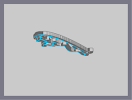Hover over the thumbnail for a full-size version.

Author RavenMac author:ravenmac cool featured n-art rated 2008-07-05 2008-07-05 5 by 144 people. \$Jaguar#RavenMac#none#00000000000000000000000000000000000000000000000000000000000000000000000000000000000000000000000000000000000000000000000000000000000000000000000000000000000000000000000000000000000000000000000000000000000000000000000000000000000000000000000000000000000000000000000000000000000000000000000000000000000000000000000000000000000000000000000000000000000000000000000000000000000000000000000000000000000000000000000000000000000000000000000000000000000000000000000000000000000000000000000000000000000000000000000000000000000000000000000000000000000000000000000000000000000000000000000000000000000000000000000000000000000000000000000000000000000000000000000000000000000000000000000000000000000000000000000000000000000000000|8^510,325,1!1^511,324!8^511,325,1!1^512,324!8^512,325,1!1^513,324!8^513,325,1!1^514,324!8^514,325,1!1^515,324!1^516,325!8^505,324,1!1^506,323!8^506,324,1!1^507,323!8^507,324,1!1^508,323!8^508,324,1!1^509,323!8^509,324,1!1^510,323!8^510,324,1!1^511,323!8^511,324,1!1^512,323!8^512,324,1!1^513,323!8^513,324,1!1^514,323!8^514,324,1!1^515,323!8^515,324,1!1^516,323!8^516,324,1!1^517,323!8^517,324,1!1^518,323!1^519,324!8^503,323,1!1^504,322!8^504,323,1!1^505,322!8^505,323,1!1^506,322!8^506,323,1!1^507,322!8^507,323,1!1^508,322!8^508,323,1!1^509,322!8^509,323,1!1^510,322!8^510,323,1!1^511,322!8^511,323,1!1^512,322!8^512,323,1!1^513,322!8^513,323,1!1^514,322!8^514,323,1!1^515,322!8^515,323,1!1^516,322!8^516,323,1!1^517,322!8^517,323,1!1^518,322!8^518,323,1!1^519,322!8^519,323,1!1^520,322!1^521,323!8^501,322,1!1^502,321!8^502,322,1!1^503,321!8^503,322,1!1^504,321!8^504,322,1!1^505,321!8^505,322,1!1^506,321!8^506,322,1!1^507,321!8^507,322,1!1^508,321!8^508,322,1!1^509,321!8^509,322,1!1^510,321!8^510,322,1!1^511,321!8^511,322,1!1^512,321!8^512,322,1!1^513,321!8^513,322,1!1^514,321!8^514,322,1!1^515,321!8^515,322,1!1^516,321!8^516,322,1!1^517,321!8^517,322,1!1^518,321!8^518,322,1!1^519,321!8^519,322,1!1^520,321!1^521,322!8^499,321,1!1^500,320!8^500,321,1!1^501,320!8^501,321,1!1^502,320!8^502,321,1!1^503,320!8^503,321,1!1^504,320!8^504,321,1!1^505,320!8^505,321,1!1^506,320!8^506,321,1!1^507,320!8^507,321,1!1^508,320!8^508,321,1!1^509,320!8^509,321,1!1^510,320!8^510,321,1!1^511,320!8^511,321,1!1^512,320!8^512,321,1!1^513,320!8^513,321,1!1^514,320!8^514,321,1!1^515,320!8^515,321,1!1^516,320!8^516,321,1!1^517,320!8^517,321,1!1^518,320!8^518,321,1!1^519,320!8^519,321,1!1^520,320!8^520,321,1!1^521,320!1^522,321!8^497,320,1!1^498,319!8^498,320,1!1^499,319!8^499,320,1!1^500,319!8^500,320,1!1^501,319!8^501,320,1!1^502,319!8^502,320,1!1^503,319!1^504,320!8^512,320,1!1^513,319!8^513,320,1!1^514,319!8^514,320,1!1^515,319!8^515,320,1!1^516,319!8^516,320,1!1^517,319!8^517,320,1!1^518,319!8^518,320,1!1^519,319!8^519,320,1!1^520,319!8^520,320,1!1^521,319!1^522,320!8^496,319,1!1^497,318!8^497,319,1!1^498,318!8^498,319,1!1^499,318!8^499,319,1!1^500,318!1^501,319!8^513,319,1!1^514,318!8^514,319,1!1^515,318!8^515,319,1!1^516,318!8^516,319,1!1^517,318!8^517,319,1!1^518,318!8^518,319,1!1^519,318!8^519,319,1!1^520,318!8^520,319,1!1^521,318!8^521,319,1!1^522,318!1^523,319!8^494,318,1!1^495,317!8^495,318,1!1^496,317!8^496,318,1!1^497,317!8^497,318,1!1^498,317!1^499,318!8^514,318,1!1^515,317!8^515,318,1!1^516,317!8^516,318,1!1^517,317!8^517,318,1!1^518,317!8^518,318,1!1^519,317!8^519,318,1!1^520,317!8^520,318,1!1^521,317!8^521,318,1!1^522,317!1^523,318!8^493,317,1!1^494,316!8^494,317,1!1^495,316!8^495,317,1!1^496,316!1^497,317!8^515,317,1!1^516,316!8^516,317,1!1^517,316!8^517,317,1!1^518,316!8^518,317,1!1^519,316!8^519,317,1!1^520,316!8^520,317,1!1^521,316!8^521,317,1!1^522,316!1^523,317!8^491,316,1!1^492,315!8^492,316,1!1^493,315!8^493,316,1!1^494,315!8^494,316,1!1^495,315!1^496,316!8^515,316,1!1^516,315!8^516,316,1!1^517,315!8^517,316,1!1^518,315!8^518,316,1!1^519,315!8^519,316,1!1^520,315!8^520,316,1!1^521,315!8^521,316,1!1^522,315!1^523,316!8^490,315,1!1^491,314!8^491,315,1!1^492,314!8^492,315,1!1^493,314!8^493,315,1!1^494,314!1^495,315!8^515,315,1!1^516,314!8^516,315,1!1^517,314!8^517,315,1!1^518,314!8^518,315,1!1^519,314!8^519,315,1!1^520,314!8^520,315,1!1^521,314!1^523,315!8^489,314,1!1^490,313!8^490,314,1!1^491,313!8^491,314,1!1^492,313!1^493,314!8^515,314,1!1^516,313!8^516,314,1!1^517,313!8^517,314,1!1^518,313!8^518,314,1!1^519,313!8^519,314,1!1^520,313!8^520,314,1!1^521,313!1^523,314!8^488,313,1!1^489,312!8^489,313,1!1^490,312!8^490,313,1!1^491,312!1^492,313!8^515,313,1!1^516,312!8^516,313,1!1^517,312!8^517,313,1!1^518,312!8^518,313,1!1^519,312!8^519,313,1!1^520,312!8^520,313,1!1^521,312!8^521,313,1!1^522,312!8^522,313,1!1^523,312!8^523,313,1!1^524,312!8^524,313,1!1^525,312!8^525,313,1!1^526,312!8^526,313,1!1^527,312!8^527,313,1!1^528,312!8^528,313,1!1^529,312!8^529,313,1!1^530,312!8^530,313,1!1^531,312!8^531,313,1!1^532,312!8^532,313,1!1^533,312!1^534,313!8^486,312,1!1^487,311!8^487,312,1!1^488,311!8^488,312,1!1^489,311!8^489,312,1!1^490,311!1^491,312!8^514,312,1!1^515,311!8^515,312,1!1^516,311!8^516,312,1!1^517,311!8^517,312,1!1^518,311!8^518,312,1!1^519,311!8^519,312,1!1^520,311!8^520,312,1!1^521,311!8^521,312,1!1^522,311!8^522,312,1!1^523,311!8^523,312,1!1^524,311!8^524,312,1!1^525,311!8^525,312,1!1^526,311!8^526,312,1!1^527,311!8^527,312,1!1^528,311!8^528,312,1!1^529,311!8^529,312,1!1^530,311!8^530,312,1!1^531,311!8^531,312,1!1^532,311!8^532,312,1!1^533,311!8^533,312,1!1^534,311!8^534,312,1!1^535,311!8^535,312,1!1^536,311!8^536,312,1!1^537,311!1^538,312!8^485,311,1!1^486,310!8^486,311,1!1^487,310!8^487,311,1!1^488,310!8^489,311,1!1^489,310!1^491,311!8^513,311,1!1^514,310!8^514,311,1!1^515,310!8^515,311,1!1^516,310!8^516,311,1!1^517,310!8^517,311,1!1^518,310!8^518,311,1!1^519,310!8^519,311,1!1^520,310!1^521,311!8^537,311,1!1^538,310!8^538,311,1!1^539,310!8^539,311,1!1^540,310!1^541,311!8^484,310,1!1^485,309!8^485,310,1!1^486,309!8^486,310,1!1^487,309!8^487,310,1!1^488,309!1^489,310!8^511,310,1!1^512,309!8^512,310,1!1^513,309!8^513,310,1!1^514,309!8^514,310,1!1^515,309!8^515,310,1!1^516,309!8^516,310,1!1^517,309!8^517,310,1!1^518,309!1^519,310!8^539,310,1!1^540,309!8^540,310,1!1^541,309!8^541,310,1!1^542,309!1^543,310!8^483,309,1!1^484,308!8^484,309,1!1^485,308!8^485,309,1!1^486,308!1^487,309!8^509,309,1!1^510,308!8^510,309,1!1^511,308!8^511,309,1!1^512,308!8^512,309,1!1^513,308!8^513,309,1!1^514,308!8^514,309,1!1^515,308!1^516,309!8^541,309,1!1^542,308!8^542,309,1!1^543,308!8^543,309,1!1^544,308!1^545,309!8^481,308,1!1^482,307!8^482,308,1!1^483,307!8^483,308,1!1^484,307!8^484,308,1!1^485,307!1^486,308!8^507,308,1!1^508,307!8^508,308,1!1^509,307!8^509,308,1!1^510,307!8^510,308,1!1^511,307!8^511,308,1!1^512,307!1^513,308!8^543,308,1!1^544,307!8^544,308,1!1^545,307!1^546,308!8^480,307,1!1^481,306!8^481,307,1!1^482,306!8^482,307,1!1^483,306!8^483,307,1!1^484,306!1^485,307!8^506,307,1!1^507,306!8^507,307,1!1^508,306!8^508,307,1!1^509,306!1^510,307!8^544,307,1!1^545,306!8^545,307,1!1^546,306!1^547,307!8^478,306,1!1^479,305!8^479,306,1!1^480,305!8^480,306,1!1^481,305!8^481,306,1!1^482,305!8^482,306,1!1^483,305!1^484,306!8^504,306,1!1^505,305!8^505,306,1!1^506,305!8^506,306,1!1^507,305!1^508,306!8^545,306,1!1^546,305!8^546,306,1!1^547,305!1^548,306!8^475,305,1!1^476,304!8^476,305,1!1^477,304!8^477,305,1!1^478,304!8^478,305,1!1^479,304!8^479,305,1!1^480,304!8^480,305,1!1^481,304!1^482,305!8^502,305,1!1^503,304!8^503,305,1!1^504,304!8^504,305,1!1^505,304!1^506,305!8^546,305,1!1^547,304!1^548,305!8^471,304,1!1^472,303!8^472,304,1!1^473,303!8^473,304,1!1^474,303!8^474,304,1!1^475,303!8^475,304,1!1^476,303!8^476,304,1!1^477,303!8^477,304,1!1^478,303!8^478,304,1!1^479,303!8^479,304,1!1^480,303!1^481,304!8^501,304,1!1^502,303!8^502,304,1!1^503,303!8^503,304,1!1^504,303!1^505,304!8^546,304,1!1^547,303!8^547,304,1!1^548,303!1^549,304!8^433,303,1!1^434,302!8^434,303,1!1^435,302!8^435,303,1!1^436,302!8^436,303,1!1^437,302!8^437,303,1!1^438,302!8^438,303,1!1^439,302!8^439,303,1!1^440,302!8^440,303,1!1^441,302!8^441,303,1!1^442,302!8^442,303,1!1^443,302!8^443,303,1!1^444,302!8^444,303,1!1^445,302!8^445,303,1!1^446,302!8^446,303,1!1^447,302!8^447,303,1!1^448,302!8^448,303,1!1^449,302!8^449,303,1!1^450,302!8^450,303,1!1^451,302!8^451,303,1!1^452,302!8^452,303,1!1^453,302!8^453,303,1!1^454,302!8^454,303,1!1^455,302!8^455,303,1!1^456,302!8^456,303,1!1^457,302!8^457,303,1!1^458,302!8^458,303,1!1^459,302!8^459,303,1!1^460,302!8^460,303,1!1^461,302!8^461,303,1!1^462,302!8^462,303,1!1^463,302!8^463,303,1!1^464,302!8^464,303,1!1^465,302!8^465,303,1!1^466,302!8^466,303,1!1^467,302!8^467,303,1!1^468,302!8^468,303,1!1^469,302!8^469,303,1!1^470,302!8^470,303,1!1^471,302!8^471,303,1!1^472,302!8^472,303,1!1^473,302!8^473,303,1!1^474,302!8^474,303,1!1^475,302!8^475,303,1!1^476,302!8^476,303,1!1^477,302!8^477,303,1!1^478,302!1^479,303!8^500,303,1!1^501,302!8^501,303,1!1^502,302!8^502,303,1!1^503,302!1^504,303!8^526,303,1!1^527,302!8^527,303,1!1^528,302!8^528,303,1!1^529,302!8^529,303,1!1^530,302!8^530,303,1!1^531,302!8^531,303,1!1^532,302!1^533,303!8^546,303,1!1^547,302!8^547,303,1!1^548,302!1^549,303!8^428,302,1!1^429,301!8^429,302,1!1^430,301!8^430,302,1!1^431,301!8^431,302,1!1^432,301!8^432,302,1!1^433,301!8^433,302,1!1^434,301!8^434,302,1!1^435,301!8^435,302,1!1^436,301!8^436,302,1!1^437,301!8^437,302,1!1^438,301!8^438,302,1!1^439,301!8^439,302,1!1^440,301!8^440,302,1!1^441,301!8^441,302,1!1^442,301!8^442,302,1!1^443,301!8^443,302,1!1^444,301!8^444,302,1!1^445,301!8^445,302,1!1^446,301!8^446,302,1!1^447,301!8^447,302,1!1^448,301!8^448,302,1!1^449,301!8^449,302,1!1^450,301!8^450,302,1!1^451,301!8^451,302,1!1^452,301!8^452,302,1!1^453,301!8^453,302,1!1^454,301!8^454,302,1!1^455,301!8^455,302,1!1^456,301!8^456,302,1!1^457,301!8^457,302,1!1^458,301!8^458,302,1!1^459,301!8^459,302,1!1^460,301!8^460,302,1!1^461,301!8^461,302,1!1^462,301!8^462,302,1!1^463,301!8^463,302,1!1^464,301!8^464,302,1!1^465,301!8^465,302,1!1^466,301!8^466,302,1!1^467,301!8^467,302,1!1^468,301!8^468,302,1!1^469,301!8^469,302,1!1^470,301!8^470,302,1!1^471,301!8^471,302,1!1^472,301!8^472,302,1!1^473,301!8^473,302,1!1^474,301!8^474,302,1!1^475,301!8^475,302,1!1^476,301!1^477,302!8^499,302,1!1^500,301!8^500,302,1!1^501,301!1^502,302!8^520,302,1!1^521,301!8^521,302,1!1^522,301!8^522,302,1!1^523,301!8^523,302,1!1^524,301!8^524,302,1!1^525,301!8^525,302,1!1^526,301!8^526,302,1!1^527,301!8^527,302,1!1^528,301!8^528,302,1!1^529,301!8^529,302,1!1^530,301!8^530,302,1!1^531,301!8^531,302,1!1^532,301!8^532,302,1!1^533,301!8^533,302,1!1^534,301!8^534,302,1!1^535,301!8^535,302,1!1^536,301!1^537,302!8^546,302,1!1^547,301!8^547,302,1!1^548,301!8^548,302,1!1^549,301!1^550,302!8^425,301,1!1^426,300!8^426,301,1!1^427,300!8^427,301,1!1^428,300!8^428,301,1!1^429,300!8^429,301,1!1^430,300!8^430,301,1!1^431,300!8^431,301,1!1^432,300!8^432,301,1!1^433,300!8^433,301,1!1^434,300!8^434,301,1!1^435,300!8^435,301,1!1^436,300!8^436,301,1!1^437,300!8^437,301,1!1^438,300!8^438,301,1!1^439,300!8^439,301,1!1^440,300!8^440,301,1!1^441,300!8^441,301,1!1^442,300!8^442,301,1!1^443,300!8^443,301,1!1^444,300!8^444,301,1!1^445,300!8^445,301,1!1^446,300!8^446,301,1!1^447,300!8^447,301,1!1^448,300!8^448,301,1!1^449,300!8^449,301,1!1^450,300!8^450,301,1!1^451,300!8^451,301,1!1^452,300!8^452,301,1!1^453,300!8^453,301,1!1^454,300!8^454,301,1!1^455,300!8^455,301,1!1^456,300!8^456,301,1!1^457,300!8^457,301,1!1^458,300!8^458,301,1!1^459,300!8^459,301,1!1^460,300!8^460,301,1!1^461,300!8^461,301,1!1^462,300!8^462,301,1!1^463,300!8^463,301,1!1^464,300!8^464,301,1!1^465,300!8^465,301,1!1^466,300!8^466,301,1!1^467,300!8^467,301,1!1^468,300!8^468,301,1!1^469,300!8^469,301,1!1^470,300!8^470,301,1!1^471,300!8^471,301,1!1^472,300!8^472,301,1!1^473,300!8^473,301,1!1^474,300!1^475,301!8^498,301,1!1^499,300!8^499,301,1!1^500,300!1^501,301!8^517,301,1!1^518,300!8^518,301,1!1^519,300!8^519,301,1!1^520,300!8^520,301,1!1^521,300!8^521,301,1!1^522,300!1^523,301!8^531,301,1!1^532,300!8^532,301,1!1^533,300!8^533,301,1!1^534,300!8^534,301,1!1^535,300!8^535,301,1!1^536,300!8^536,301,1!1^537,300!8^537,301,1!1^538,300!1^539,301!8^545,301,1!1^546,300!8^546,301,1!1^547,300!8^547,301,1!1^548,300!8^548,301,1!1^549,300!1^550,301!8^423,300,1!1^424,299!8^424,300,1!1^425,299!8^425,300,1!1^426,299!8^426,300,1!1^427,299!8^427,300,1!1^428,299!8^428,300,1!1^429,299!8^429,300,1!1^430,299!8^430,300,1!1^431,299!8^431,300,1!1^432,299!8^432,300,1!1^433,299!8^433,300,1!1^434,299!8^434,300,1!1^435,299!8^435,300,1!1^436,299!8^436,300,1!1^437,299!8^437,300,1!1^438,299!8^438,300,1!1^439,299!8^439,300,1!1^440,299!8^440,300,1!1^441,299!8^441,300,1!1^442,299!8^442,300,1!1^443,299!8^443,300,1!1^444,299!8^444,300,1!1^445,299!8^445,300,1!1^446,299!8^446,300,1!1^447,299!8^447,300,1!1^448,299!8^448,300,1!1^449,299!8^449,300,1!1^450,299!8^450,300,1!1^451,299!8^451,300,1!1^452,299!8^452,300,1!1^453,299!8^453,300,1!1^454,299!8^454,300,1!1^455,299!8^455,300,1!1^456,299!8^456,300,1!1^457,299!8^457,300,1!1^458,299!8^458,300,1!1^459,299!8^459,300,1!1^460,299!8^460,300,1!1^461,299!8^461,300,1!1^462,299!8^462,300,1!1^463,299!8^463,300,1!1^464,299!8^464,300,1!1^465,299!8^465,300,1!1^466,299!8^466,300,1!1^467,299!8^467,300,1!1^468,299!8^468,300,1!1^469,299!8^469,300,1!1^470,299!1^471,300!8^497,300,1!1^498,299!8^498,300,1!1^499,299!1^500,300!8^514,300,1!1^515,299!8^515,300,1!1^516,299!8^516,300,1!1^517,299!8^517,300,1!1^518,299!1^519,300!8^535,300,1!1^536,299!8^536,300,1!1^537,299!8^537,300,1!1^538,299!8^538,300,1!1^539,299!8^539,300,1!1^540,299!8^540,300,1!1^541,299!1^542,300!8^544,300,1!1^545,299!8^545,300,1!1^546,299!8^546,300,1!1^547,299!8^547,300,1!1^548,299!8^548,300,1!1^549,299!1^550,300!8^421,299,1!1^422,298!8^422,299,1!1^423,298!8^423,299,1!1^424,298!8^424,299,1!1^425,298!8^425,299,1!1^426,298!8^426,299,1!1^427,298!8^427,299,1!1^428,298!8^428,299,1!1^429,298!8^429,299,1!1^430,298!8^430,299,1!1^431,298!8^431,299,1!1^432,298!8^432,299,1!1^433,298!8^433,299,1!1^434,298!8^434,299,1!1^435,298!8^435,299,1!1^436,298!8^436,299,1!1^437,298!8^437,299,1!1^438,298!8^438,299,1!1^439,298!8^439,299,1!1^440,298!8^440,299,1!1^441,298!8^441,299,1!1^442,298!8^442,299,1!1^443,298!8^443,299,1!1^444,298!8^444,299,1!1^445,298!8^445,299,1!1^446,298!8^446,299,1!1^447,298!8^447,299,1!1^448,298!8^448,299,1!1^449,298!8^449,299,1!1^450,298!8^450,299,1!1^451,298!8^451,299,1!1^452,298!8^452,299,1!1^453,298!8^453,299,1!1^454,298!8^454,299,1!1^455,298!8^455,299,1!1^456,298!8^456,299,1!1^457,298!8^457,299,1!1^458,298!8^458,299,1!1^459,298!8^459,299,1!1^460,298!8^460,299,1!1^461,298!8^461,299,1!1^462,298!8^462,299,1!1^463,298!1^464,299!8^497,299,1!1^498,298!1^499,299!8^512,299,1!1^513,298!8^513,299,1!1^514,298!8^514,299,1!1^515,298!1^516,299!8^537,299,1!1^538,298!8^538,299,1!1^539,298!8^539,299,1!1^540,298!8^540,299,1!1^541,298!8^541,299,1!1^542,298!8^542,299,1!1^543,298!8^543,299,1!1^544,298!8^544,299,1!1^545,298!8^545,299,1!1^546,298!8^546,299,1!1^547,298!8^547,299,1!1^548,298!1^549,299!8^419,298,1!1^420,297!8^420,298,1!1^421,297!8^421,298,1!1^422,297!8^422,298,1!1^423,297!8^423,298,1!1^424,297!8^424,298,1!1^425,297!8^425,298,1!1^426,297!8^426,298,1!1^427,297!8^427,298,1!1^428,297!8^428,298,1!1^429,297!8^429,298,1!1^430,297!8^430,298,1!1^431,297!8^431,298,1!1^432,297!1^433,298!8^496,298,1!1^497,297!1^498,298!8^510,298,1!1^511,297!8^511,298,1!1^512,297!8^512,298,1!1^513,297!1^514,298!8^539,298,1!1^540,297!8^540,298,1!1^541,297!8^541,298,1!1^542,297!8^542,298,1!1^543,297!8^543,298,1!1^544,297!8^544,298,1!1^545,297!8^545,298,1!1^546,297!8^546,298,1!1^547,297!1^548,298!8^417,297,1!1^418,296!8^418,297,1!1^419,296!8^419,297,1!1^420,296!8^420,297,1!1^421,296!8^421,297,1!1^422,296!8^422,297,1!1^423,296!8^423,297,1!1^424,296!8^424,297,1!1^425,296!8^425,297,1!1^426,296!8^426,297,1!1^427,296!8^427,297,1!1^428,296!1^429,297!8^495,297,1!1^496,296!8^496,297,1!1^497,296!1^498,297!8^508,297,1!1^509,296!8^509,297,1!1^510,296!8^510,297,1!1^511,296!1^512,297!8^416,296,1!1^417,295!8^417,296,1!1^418,295!8^418,296,1!1^419,295!8^419,296,1!1^420,295!8^420,296,1!1^421,295!8^421,296,1!1^422,295!8^422,296,1!1^423,295!8^423,296,1!1^424,295!1^425,296!8^494,296,1!1^495,295!1^496,296!8^506,296,1!1^507,295!8^507,296,1!1^508,295!8^508,296,1!1^509,295!1^510,296!8^415,295,1!1^416,294!8^416,295,1!1^417,294!8^417,295,1!1^418,294!8^418,295,1!1^419,294!8^419,295,1!1^420,294!8^420,295,1!1^421,294!8^421,295,1!1^422,294!1^423,295!8^493,295,1!1^494,294!8^494,295,1!1^495,294!1^496,295!8^504,295,1!1^505,294!8^505,295,1!1^506,294!8^506,295,1!1^507,294!1^508,295!8^413,294,1!1^414,293!8^414,294,1!1^415,293!8^415,294,1!1^416,293!8^416,294,1!1^417,293!8^417,294,1!1^418,293!8^418,294,1!1^419,293!8^419,294,1!1^420,293!1^421,294!8^492,294,1!1^493,293!8^493,294,1!1^494,293!1^495,294!8^503,294,1!1^504,293!8^504,294,1!1^505,293!1^506,294!8^412,293,1!1^413,292!8^413,293,1!1^414,292!8^414,293,1!1^415,292!8^415,293,1!1^416,292!8^416,293,1!1^417,292!8^417,293,1!1^418,292!1^419,293!8^491,293,1!1^492,292!8^492,293,1!1^493,292!1^494,293!8^501,293,1!1^502,292!8^502,293,1!1^503,292!1^504,293!8^411,292,1!1^412,291!8^412,292,1!1^413,291!8^413,292,1!1^414,291!8^414,292,1!1^415,291!8^415,292,1!1^416,291!8^416,292,1!1^417,291!1^418,292!8^490,292,1!1^491,291!8^491,292,1!1^492,291!1^493,292!8^499,292,1!1^500,291!8^500,292,1!1^501,291!8^501,292,1!1^502,291!1^503,292!8^410,291,1!1^411,290!8^411,291,1!1^412,290!8^412,291,1!1^413,290!8^413,291,1!1^414,290!8^414,291,1!1^415,290!1^416,291!8^489,291,1!1^490,290!1^491,291!8^497,291,1!1^498,290!8^498,291,1!1^499,290!8^499,291,1!1^500,290!1^501,291!8^410,290,1!1^411,289!8^411,290,1!1^412,289!8^412,290,1!1^413,289!8^413,290,1!1^414,289!1^415,290!8^487,290,1!1^488,289!8^488,290,1!1^489,289!1^490,290!8^495,290,1!1^496,289!8^496,290,1!1^497,289!8^497,290,1!1^498,289!1^499,290!8^409,289,1!1^410,288!8^410,289,1!1^411,288!8^411,289,1!1^412,288!8^412,289,1!1^413,288!1^414,289!8^484,289,1!1^485,288!8^485,289,1!1^486,288!8^486,289,1!1^487,288!8^487,289,1!1^488,288!1^489,289!8^494,289,1!1^495,288!8^495,289,1!1^496,288!8^496,289,1!1^497,288!1^498,289!8^408,288,1!1^409,287!8^409,288,1!1^410,287!8^410,288,1!1^411,287!8^411,288,1!1^412,287!1^413,288!8^479,288,1!1^480,287!8^480,288,1!1^481,287!8^481,288,1!1^482,287!8^482,288,1!1^483,287!8^483,288,1!1^484,287!8^484,288,1!1^485,287!1^486,288!8^492,288,1!1^493,287!8^493,288,1!1^494,287!8^494,288,1!1^495,287!1^496,288!8^407,287,1!1^408,286!8^408,287,1!1^409,286!8^409,287,1!1^410,286!8^410,287,1!1^411,286!1^412,287!8^462,287,1!1^463,286!8^463,287,1!1^464,286!8^464,287,1!1^465,286!8^465,287,1!1^466,286!8^466,287,1!1^467,286!8^467,287,1!1^468,286!8^468,287,1!1^469,286!8^469,287,1!1^470,286!8^470,287,1!1^471,286!8^471,287,1!1^472,286!8^472,287,1!1^473,286!8^473,287,1!1^474,286!8^474,287,1!1^475,286!8^475,287,1!1^476,286!8^476,287,1!1^477,286!8^477,287,1!1^478,286!8^478,287,1!1^479,286!8^479,287,1!1^480,286!1^481,287!8^490,287,1!1^491,286!8^491,287,1!1^492,286!8^492,287,1!1^493,286!1^494,287!8^407,286,1!1^408,285!8^408,286,1!1^409,285!8^409,286,1!1^410,285!1^411,286!8^454,286,1!1^455,285!8^455,286,1!1^456,285!8^456,286,1!1^457,285!8^457,286,1!1^458,285!8^458,286,1!1^459,285!8^459,286,1!1^460,285!8^460,286,1!1^461,285!8^461,286,1!1^462,285!8^462,286,1!1^463,285!8^463,286,1!1^464,285!8^464,286,1!1^465,285!8^465,286,1!1^466,285!8^466,286,1!1^467,285!8^467,286,1!1^468,285!8^468,286,1!1^469,285!8^469,286,1!1^470,285!8^470,286,1!1^471,285!8^471,286,1!1^472,285!1^473,286!8^489,286,1!1^490,285!8^490,286,1!1^491,285!8^491,286,1!1^492,285!1^493,286!8^406,285,1!1^407,284!8^407,285,1!1^408,284!8^408,285,1!1^409,284!1^410,285!8^452,285,1!1^453,284!8^453,285,1!1^454,284!8^454,285,1!1^455,284!8^455,285,1!1^456,284!8^456,285,1!1^457,284!8^457,285,1!1^458,284!8^458,285,1!1^459,284!8^459,285,1!1^460,284!8^460,285,1!1^461,284!8^461,285,1!1^462,284!8^462,285,1!1^463,284!8^463,285,1!1^464,284!1^465,285!8^487,285,1!1^488,284!8^488,285,1!1^489,284!8^489,285,1!1^490,284!1^491,285!8^405,284,1!1^406,283!8^406,284,1!1^407,283!8^407,284,1!1^408,283!1^409,284!8^450,284,1!1^451,283!8^451,284,1!1^452,283!8^452,284,1!1^453,283!8^453,284,1!1^454,283!8^454,284,1!1^455,283!8^455,284,1!1^456,283!8^456,284,1!1^457,283!8^457,284,1!1^458,283!8^458,284,1!1^459,283!1^460,284!8^485,284,1!1^486,283!8^486,284,1!1^487,283!8^487,284,1!1^488,283!1^489,284!8^405,283,1!1^406,282!8^406,283,1!1^407,282!1^408,283!8^449,283,1!1^450,282!8^450,283,1!1^451,282!8^451,283,1!1^452,282!8^452,283,1!1^453,282!8^453,283,1!1^454,282!8^454,283,1!1^455,282!8^455,283,1!1^456,282!1^457,283!8^483,283,1!1^484,282!8^484,283,1!1^485,282!8^485,283,1!1^486,282!1^487,283!8^404,282,1!1^405,281!8^405,282,1!1^406,281!1^407,282!8^448,282,1!1^449,281!8^449,282,1!1^450,281!8^450,282,1!1^451,281!8^451,282,1!1^452,281!8^452,282,1!1^453,281!8^453,282,1!1^454,281!1^455,282!8^481,282,1!1^482,281!8^482,282,1!1^483,281!8^483,282,1!1^484,281!8^484,282,1!1^485,281!1^486,282!8^403,281,1!1^404,280!8^404,281,1!1^405,280!8^405,281,1!1^406,280!1^407,281!8^447,281,1!1^448,280!8^448,281,1!1^449,280!8^449,281,1!1^450,280!8^450,281,1!1^451,280!8^451,281,1!1^452,280!8^452,281,1!1^453,280!1^454,281!8^479,281,1!1^480,280!8^480,281,1!1^481,280!8^481,281,1!1^482,280!8^482,281,1!1^483,280!1^484,281!8^403,280,1!1^404,279!8^404,280,1!1^405,279!1^406,280!8^446,280,1!1^447,279!8^447,280,1!1^448,279!8^448,280,1!1^449,279!8^449,280,1!1^450,279!8^450,280,1!1^451,279!1^452,280!8^477,280,1!1^478,279!8^478,280,1!1^479,279!8^479,280,1!1^480,279!8^480,280,1!1^481,279!1^482,280!8^402,279,1!1^403,278!8^403,279,1!1^404,278!1^405,279!8^446,279,1!1^447,278!8^447,279,1!1^448,278!8^448,279,1!1^449,278!8^449,279,1!1^450,278!1^451,279!8^475,279,1!1^476,278!8^476,279,1!1^477,278!8^477,279,1!1^478,278!8^478,279,1!1^479,278!1^480,279!8^402,278,1!1^403,277!8^403,278,1!1^404,277!1^405,278!8^445,278,1!1^446,277!8^446,278,1!1^447,277!8^447,278,1!1^448,277!8^448,278,1!1^449,277!1^450,278!8^473,278,1!1^474,277!8^474,278,1!1^475,277!8^475,278,1!1^476,277!8^476,278,1!1^477,277!1^478,278!8^401,277,1!1^402,276!8^402,277,1!1^403,276!1^404,277!8^445,277,1!1^446,276!8^446,277,1!1^447,276!8^447,277,1!1^448,276!1^449,277!8^471,277,1!1^472,276!8^472,277,1!1^473,276!8^473,277,1!1^474,276!8^474,277,1!1^475,276!1^476,277!8^400,276,1!1^401,275!8^401,276,1!1^402,275!1^403,276!8^444,276,1!1^445,275!8^445,276,1!1^446,275!8^446,276,1!1^447,275!1^448,276!8^469,276,1!1^470,275!8^470,276,1!1^471,275!8^471,276,1!1^472,275!8^472,276,1!1^473,275!8^473,276,1!1^474,275!1^475,276!8^400,275,1!1^401,274!8^401,275,1!1^402,274!1^403,275!8^444,275,1!1^445,274!8^445,275,1!1^446,274!8^446,275,1!1^447,274!1^448,275!8^467,275,1!1^468,274!8^468,275,1!1^469,274!8^469,275,1!1^470,274!8^470,275,1!1^471,274!8^471,275,1!1^472,274!1^473,275!8^399,274,1!1^400,273!8^400,274,1!1^401,273!1^402,274!8^443,274,1!1^444,273!8^444,274,1!1^445,273!8^445,274,1!1^446,273!1^447,274!8^465,274,1!1^466,273!8^466,274,1!1^467,273!8^467,274,1!1^468,273!8^468,274,1!1^469,273!8^469,274,1!1^470,273!1^471,274!8^398,273,1!1^399,272!8^399,273,1!1^400,272!8^400,273,1!1^401,272!1^402,273!8^443,273,1!1^444,272!8^444,273,1!1^445,272!1^446,273!8^463,273,1!1^464,272!8^464,273,1!1^465,272!8^465,273,1!1^466,272!8^466,273,1!1^467,272!8^467,273,1!1^468,272!1^469,273!8^398,272,1!1^399,271!8^399,272,1!1^400,271!1^401,272!8^442,272,1!1^443,271!8^443,272,1!1^444,271!1^445,272!8^462,272,1!1^463,271!8^463,272,1!1^464,271!8^464,272,1!1^465,271!8^465,272,1!1^466,271!1^467,272!8^262,271,1!1^263,270!8^263,271,1!1^264,270!8^264,271,1!1^265,270!1^266,271!8^396,271,1!1^397,270!8^397,271,1!1^398,270!8^398,271,1!1^399,270!1^400,271!8^442,271,1!1^443,270!8^443,271,1!1^444,270!1^445,271!8^460,271,1!1^461,270!8^461,271,1!1^462,270!8^462,271,1!1^463,270!8^463,271,1!1^464,270!1^465,271!8^259,270,1!1^260,269!8^260,270,1!1^261,269!8^261,270,1!1^262,269!8^262,270,1!1^263,269!8^263,270,1!1^264,269!8^264,270,1!1^265,269!8^265,270,1!1^266,269!8^266,270,1!1^267,269!1^268,270!8^395,270,1!1^396,269!8^396,270,1!1^397,269!8^397,270,1!1^398,269!8^398,270,1!1^399,269!1^400,270!8^441,270,1!1^442,269!8^442,270,1!1^443,269!1^444,270!8^458,270,1!1^459,269!8^459,270,1!1^460,269!8^460,270,1!1^461,269!8^461,270,1!1^462,269!1^463,270!8^258,269,1!1^259,268!8^259,269,1!1^260,268!8^260,269,1!1^261,268!8^261,269,1!1^262,268!8^262,269,1!1^263,268!1^264,269!8^265,269,1!1^266,268!8^266,269,1!1^267,268!8^267,269,1!1^268,268!8^268,269,1!1^269,268!1^270,269!8^306,269,1!1^307,268!8^307,269,1!1^308,268!8^308,269,1!1^309,268!8^309,269,1!1^310,268!8^310,269,1!1^311,268!8^311,269,1!1^312,268!8^312,269,1!1^313,268!8^313,269,1!1^314,268!8^314,269,1!1^315,268!8^315,269,1!1^316,268!8^316,269,1!1^317,268!8^317,269,1!1^318,268!8^318,269,1!1^319,268!1^320,269!8^392,269,1!1^393,268!8^393,269,1!1^394,268!8^394,269,1!1^395,268!8^395,269,1!1^396,268!8^396,269,1!1^397,268!8^397,269,1!1^398,268!1^399,269!8^441,269,1!1^442,268!1^443,269!8^456,269,1!1^457,268!8^457,269,1!1^458,268!8^458,269,1!1^459,268!8^459,269,1!1^460,268!1^461,269!8^257,268,1!1^258,267!8^258,268,1!1^259,267!8^259,268,1!1^260,267!8^260,268,1!1^261,267!1^262,268!8^267,268,1!1^268,267!8^268,268,1!1^269,267!8^269,268,1!1^270,267!1^271,268!8^301,268,1!1^302,267!8^302,268,1!1^303,267!8^303,268,1!1^304,267!8^304,268,1!1^305,267!8^305,268,1!1^306,267!8^306,268,1!1^307,267!8^307,268,1!1^308,267!8^308,268,1!1^309,267!8^309,268,1!1^310,267!8^310,268,1!1^311,267!8^311,268,1!1^312,267!8^312,268,1!1^313,267!8^313,268,1!1^314,267!8^314,268,1!1^315,267!8^315,268,1!1^316,267!8^316,268,1!1^317,267!8^317,268,1!1^318,267!8^318,268,1!1^319,267!8^319,268,1!1^320,267!8^320,268,1!1^321,267!8^321,268,1!1^322,267!1^323,268!8^349,268,1!1^350,267!8^350,268,1!1^351,267!8^351,268,1!1^352,267!8^352,268,1!1^353,267!8^353,268,1!1^354,267!8^354,268,1!1^355,267!8^355,268,1!1^356,267!8^356,268,1!1^357,267!8^357,268,1!1^358,267!8^358,268,1!1^359,267!8^359,268,1!1^360,267!8^360,268,1!1^361,267!8^361,268,1!1^362,267!8^362,268,1!1^363,267!8^363,268,1!1^364,267!8^364,268,1!1^365,267!8^365,268,1!1^366,267!8^366,268,1!1^367,267!8^367,268,1!1^368,267!8^368,268,1!1^369,267!8^369,268,1!1^370,267!8^370,268,1!1^371,267!8^371,268,1!1^372,267!8^372,268,1!1^373,267!8^373,268,1!1^374,267!8^374,268,1!1^375,267!1^376,268!8^390,268,1!1^391,267!8^391,268,1!1^392,267!8^392,268,1!1^393,267!8^393,268,1!1^394,267!8^394,268,1!1^395,267!8^395,268,1!1^396,267!8^396,268,1!1^397,267!1^398,268!8^440,268,1!1^441,267!1^442,268!8^454,268,1!1^455,267!8^455,268,1!1^456,267!8^456,268,1!1^457,267!8^457,268,1!1^458,267!1^459,268!8^256,267,1!1^257,266!8^257,267,1!1^258,266!8^258,267,1!1^259,266!8^259,267,1!1^260,266!1^261,267!8^268,267,1!1^269,266!8^269,267,1!1^270,266!8^270,267,1!1^271,266!1^272,267!8^298,267,1!1^299,266!8^299,267,1!1^300,266!8^300,267,1!1^301,266!8^301,267,1!1^302,266!8^302,267,1!1^303,266!8^303,267,1!1^304,266!8^304,267,1!1^305,266!8^305,267,1!1^306,266!8^306,267,1!1^307,266!8^307,267,1!1^308,266!8^308,267,1!1^309,266!8^309,267,1!1^310,266!8^310,267,1!1^311,266!8^311,267,1!1^312,266!8^312,267,1!1^313,266!8^313,267,1!1^314,266!8^314,267,1!1^315,266!8^315,267,1!1^316,266!8^316,267,1!1^317,266!8^317,267,1!1^318,266!8^318,267,1!1^319,266!8^319,267,1!1^320,266!8^320,267,1!1^321,266!8^321,267,1!1^322,266!8^322,267,1!1^323,266!8^323,267,1!1^324,266!8^324,267,1!1^325,266!1^326,267!8^342,267,1!1^343,266!8^343,267,1!1^344,266!8^344,267,1!1^345,266!8^345,267,1!1^346,266!8^346,267,1!1^347,266!8^347,267,1!1^348,266!8^348,267,1!1^349,266!8^349,267,1!1^350,266!8^350,267,1!1^351,266!8^351,267,1!1^352,266!8^352,267,1!1^353,266!8^353,267,1!1^354,266!8^354,267,1!1^355,266!8^355,267,1!1^356,266!8^356,267,1!1^357,266!8^357,267,1!1^358,266!8^358,267,1!1^359,266!8^359,267,1!1^360,266!8^360,267,1!1^361,266!8^361,267,1!1^362,266!8^362,267,1!1^363,266!8^363,267,1!1^364,266!8^364,267,1!1^365,266!8^365,267,1!1^366,266!8^366,267,1!1^367,266!8^367,267,1!1^368,266!8^368,267,1!1^369,266!8^369,267,1!1^370,266!8^370,267,1!1^371,266!8^371,267,1!1^372,266!8^372,267,1!1^373,266!8^373,267,1!1^374,266!8^374,267,1!1^375,266!8^375,267,1!1^376,266!8^376,267,1!1^377,266!8^377,267,1!1^378,266!8^378,267,1!1^379,266!8^379,267,1!1^380,266!8^380,267,1!1^381,266!8^381,267,1!1^382,266!8^382,267,1!1^383,266!8^383,267,1!1^384,266!8^384,267,1!1^385,266!8^385,267,1!1^386,266!8^386,267,1!1^387,266!8^387,267,1!1^388,266!8^388,267,1!1^389,266!8^389,267,1!1^390,266!8^390,267,1!1^391,266!8^391,267,1!1^392,266!8^392,267,1!1^393,266!8^393,267,1!1^394,266!8^394,267,1!1^395,266!8^395,267,1!1^396,266!1^397,267!8^439,267,1!1^440,266!1^441,267!8^452,267,1!1^453,266!8^453,267,1!1^454,266!8^454,267,1!1^455,266!8^455,267,1!1^456,266!1^457,267!8^256,266,1!1^257,265!8^257,266,1!1^258,265!8^258,266,1!1^259,265!1^260,266!8^269,266,1!1^270,265!8^270,266,1!1^271,265!8^271,266,1!1^272,265!1^273,266!8^295,266,1!1^296,265!8^296,266,1!1^297,265!8^297,266,1!1^298,265!8^298,266,1!1^299,265!8^299,266,1!1^300,265!8^300,266,1!1^301,265!8^301,266,1!1^302,265!8^302,266,1!1^303,265!8^303,266,1!1^304,265!8^304,266,1!1^305,265!8^305,266,1!1^306,265!8^306,266,1!1^307,265!8^307,266,1!1^308,265!8^308,266,1!1^309,265!8^309,266,1!1^310,265!8^310,266,1!1^311,265!8^311,266,1!1^312,265!8^312,266,1!1^313,265!8^313,266,1!1^314,265!8^314,266,1!1^315,265!8^315,266,1!1^316,265!8^316,266,1!1^317,265!8^317,266,1!1^318,265!8^318,266,1!1^319,265!8^319,266,1!1^320,265!8^320,266,1!1^321,265!8^321,266,1!1^322,265!8^322,266,1!1^323,265!8^323,266,1!1^324,265!8^324,266,1!1^325,265!8^325,266,1!1^326,265!1^327,266!8^337,266,1!1^338,265!8^338,266,1!1^339,265!8^339,266,1!1^340,265!8^340,266,1!1^341,265!8^341,266,1!1^342,265!8^342,266,1!1^343,265!8^343,266,1!1^344,265!8^344,266,1!1^345,265!8^345,266,1!1^346,265!8^346,266,1!1^347,265!8^347,266,1!1^348,265!8^348,266,1!1^349,265!8^349,266,1!1^350,265!8^350,266,1!1^351,265!8^351,266,1!1^352,265!8^352,266,1!1^353,265!8^353,266,1!1^354,265!8^354,266,1!1^355,265!8^355,266,1!1^356,265!8^356,266,1!1^357,265!8^357,266,1!1^358,265!8^358,266,1!1^359,265!8^359,266,1!1^360,265!8^360,266,1!1^361,265!8^361,266,1!1^362,265!8^362,266,1!1^363,265!8^363,266,1!1^364,265!8^364,266,1!1^365,265!8^365,266,1!1^366,265!8^366,266,1!1^367,265!8^367,266,1!1^368,265!8^368,266,1!1^369,265!8^369,266,1!1^370,265!8^370,266,1!1^371,265!8^371,266,1!1^372,265!8^372,266,1!1^373,265!8^373,266,1!1^374,265!8^374,266,1!1^375,265!8^375,266,1!1^376,265!8^376,266,1!1^377,265!8^377,266,1!1^378,265!8^378,266,1!1^379,265!8^379,266,1!1^380,265!8^380,266,1!1^381,265!8^381,266,1!1^382,265!8^382,266,1!1^383,265!8^383,266,1!1^384,265!8^384,266,1!1^385,265!8^385,266,1!1^386,265!8^386,266,1!1^387,265!8^387,266,1!1^388,265!8^388,266,1!1^389,265!8^389,266,1!1^390,265!8^390,266,1!1^391,265!8^391,266,1!1^392,265!8^392,266,1!1^393,265!8^393,266,1!1^394,265!8^394,266,1!1^395,265!8^395,266,1!1^396,265!1^397,266!8^450,266,1!1^451,265!8^451,266,1!1^452,265!8^452,266,1!1^453,265!8^453,266,1!1^454,265!1^455,266!8^256,265,1!1^257,264!8^257,265,1!1^258,264!8^258,265,1!1^259,264!1^260,265!8^270,265,1!1^271,264!8^271,265,1!1^272,264!1^273,265!8^292,265,1!1^293,264!8^293,265,1!1^294,264!8^294,265,1!1^295,264!8^295,265,1!1^296,264!8^296,265,1!1^297,264!8^297,265,1!1^298,264!8^298,265,1!1^299,264!8^299,265,1!1^300,264!8^300,265,1!1^301,264!8^301,265,1!1^302,264!8^302,265,1!1^303,264!8^303,265,1!1^304,264!8^304,265,1!1^305,264!8^305,265,1!1^306,264!8^306,265,1!1^307,264!8^307,265,1!1^308,264!8^308,265,1!1^309,264!8^309,265,1!1^310,264!8^310,265,1!1^311,264!8^311,265,1!1^312,264!8^312,265,1!1^313,264!8^313,265,1!1^314,264!8^314,265,1!1^315,264!8^315,265,1!1^316,264!8^316,265,1!1^317,264!8^317,265,1!1^318,264!8^318,265,1!1^319,264!8^319,265,1!1^320,264!8^320,265,1!1^321,264!8^321,265,1!1^322,264!8^322,265,1!1^323,264!8^323,265,1!1^324,264!8^324,265,1!1^325,264!8^325,265,1!1^326,264!8^326,265,1!1^327,264!1^328,265!8^334,265,1!1^335,264!8^335,265,1!1^336,264!8^336,265,1!1^337,264!8^337,265,1!1^338,264!8^338,265,1!1^339,264!8^339,265,1!1^340,264!8^340,265,1!1^341,264!8^341,265,1!1^342,264!8^342,265,1!1^343,264!8^343,265,1!1^344,264!8^344,265,1!1^345,264!8^345,265,1!1^346,264!8^346,265,1!1^347,264!8^347,265,1!1^348,264!8^348,265,1!1^349,264!8^349,265,1!1^350,264!8^350,265,1!1^351,264!8^351,265,1!1^352,264!8^352,265,1!1^353,264!8^353,265,1!1^354,264!8^354,265,1!1^355,264!8^355,265,1!1^356,264!1^357,265!8^390,265,1!1^391,264!8^391,265,1!1^392,264!8^392,265,1!1^393,264!8^393,265,1!1^394,264!8^394,265,1!1^395,264!1^396,265!8^448,265,1!1^449,264!8^449,265,1!1^450,264!8^450,265,1!1^451,264!8^451,265,1!1^452,264!1^453,265!8^256,264,1!1^257,263!8^257,264,1!1^258,263!8^258,264,1!1^259,263!1^260,264!8^271,264,1!1^272,263!8^272,264,1!1^273,263!1^274,264!8^290,264,1!1^291,263!8^291,264,1!1^292,263!8^292,264,1!1^293,263!8^293,264,1!1^294,263!8^294,264,1!1^295,263!8^295,264,1!1^296,263!8^296,264,1!1^297,263!8^297,264,1!1^298,263!8^298,264,1!1^299,263!8^299,264,1!1^300,263!8^300,264,1!1^301,263!8^301,264,1!1^302,263!8^302,264,1!1^303,263!8^303,264,1!1^304,263!8^304,264,1!1^305,263!8^305,264,1!1^306,263!8^306,264,1!1^307,263!8^307,264,1!1^308,263!8^308,264,1!1^309,263!8^309,264,1!1^310,263!8^310,264,1!1^311,263!8^311,264,1!1^312,263!8^312,264,1!1^313,263!8^313,264,1!1^314,263!8^314,264,1!1^315,263!8^315,264,1!1^316,263!8^316,264,1!1^317,263!8^317,264,1!1^318,263!8^318,264,1!1^319,263!8^319,264,1!1^320,263!8^320,264,1!1^321,263!8^321,264,1!1^322,263!8^322,264,1!1^323,263!8^323,264,1!1^324,263!8^324,264,1!1^325,263!8^325,264,1!1^326,263!8^326,264,1!1^327,263!8^327,264,1!1^328,263!1^329,264!8^330,264,1!1^331,263!8^331,264,1!1^332,263!8^332,264,1!1^333,263!8^333,264,1!1^334,263!8^334,264,1!1^335,263!8^335,264,1!1^336,263!8^336,264,1!1^337,263!8^337,264,1!1^338,263!8^338,264,1!1^339,263!8^339,264,1!1^340,263!8^340,264,1!1^341,263!8^341,264,1!1^342,263!8^342,264,1!1^343,263!8^343,264,1!1^344,263!8^344,264,1!1^345,263!8^345,264,1!1^346,263!8^346,264,1!1^347,263!8^347,264,1!1^348,263!8^348,264,1!1^349,263!1^350,264!8^390,264,1!1^391,263!8^391,264,1!1^392,263!8^392,264,1!1^393,263!8^393,264,1!1^394,263!1^395,264!8^446,264,1!1^447,263!8^447,264,1!1^448,263!8^448,264,1!1^449,263!8^449,264,1!1^450,263!1^451,264!8^256,263,1!1^257,262!8^257,263,1!1^258,262!8^258,263,1!1^259,262!1^260,263!8^271,263,1!1^272,262!8^272,263,1!1^273,262!1^274,263!8^288,263,1!1^289,262!8^289,263,1!1^290,262!8^290,263,1!1^291,262!8^291,263,1!1^292,262!8^292,263,1!1^293,262!8^293,263,1!1^294,262!8^294,263,1!1^295,262!8^295,263,1!1^296,262!8^296,263,1!1^297,262!8^297,263,1!1^298,262!8^298,263,1!1^299,262!8^299,263,1!1^300,262!8^300,263,1!1^301,262!8^301,263,1!1^302,262!8^302,263,1!1^303,262!8^303,263,1!1^304,262!8^304,263,1!1^305,262!1^306,263!8^313,263,1!1^314,262!8^314,263,1!1^315,262!8^315,263,1!1^316,262!8^316,263,1!1^317,262!8^317,263,1!1^318,262!8^318,263,1!1^319,262!8^319,263,1!1^320,262!8^320,263,1!1^321,262!8^321,263,1!1^322,262!8^322,263,1!1^323,262!8^323,263,1!1^324,262!8^324,263,1!1^325,262!8^325,263,1!1^326,262!8^326,263,1!1^327,262!8^327,263,1!1^328,262!8^328,263,1!1^329,262!8^329,263,1!1^330,262!8^330,263,1!1^331,262!8^331,263,1!1^332,262!8^332,263,1!1^333,262!8^333,263,1!1^334,262!8^334,263,1!1^335,262!8^335,263,1!1^336,262!8^336,263,1!1^337,262!8^337,263,1!1^338,262!8^338,263,1!1^339,262!8^339,263,1!1^340,262!8^340,263,1!1^341,262!8^341,263,1!1^342,262!8^342,263,1!1^343,262!8^343,263,1!1^344,262!8^344,263,1!1^345,262!1^346,263!8^390,263,1!1^391,262!8^391,263,1!1^392,262!8^392,263,1!1^393,262!1^394,263!8^444,263,1!1^445,262!8^445,263,1!1^446,262!8^446,263,1!1^447,262!8^447,263,1!1^448,262!1^449,263!8^256,262,1!1^257,261!8^257,262,1!1^258,261!8^258,262,1!1^259,261!1^260,262!8^270,262,1!1^271,261!8^271,262,1!1^272,261!8^272,262,1!1^273,261!1^274,262!8^286,262,1!1^287,261!8^287,262,1!1^288,261!8^288,262,1!1^289,261!8^289,262,1!1^290,261!8^290,262,1!1^291,261!8^291,262,1!1^292,261!8^292,262,1!1^293,261!8^293,262,1!1^294,261!8^294,262,1!1^295,261!8^295,262,1!1^296,261!8^296,262,1!1^297,261!8^297,262,1!1^298,261!8^298,262,1!1^299,261!8^299,262,1!1^300,261!1^301,262!8^317,262,1!1^318,261!8^318,262,1!1^319,261!8^319,262,1!1^320,261!8^320,262,1!1^321,261!8^321,262,1!1^322,261!8^322,262,1!1^323,261!8^323,262,1!1^324,261!8^324,262,1!1^325,261!8^325,262,1!1^326,261!8^326,262,1!1^327,261!8^327,262,1!1^328,261!8^328,262,1!1^329,261!8^329,262,1!1^330,261!8^330,262,1!1^331,261!8^331,262,1!1^332,261!8^332,262,1!1^333,261!8^333,262,1!1^334,261!8^334,262,1!1^335,261!8^335,262,1!1^336,261!8^336,262,1!1^337,261!8^337,262,1!1^338,261!8^338,262,1!1^339,261!8^339,262,1!1^340,261!8^340,262,1!1^341,261!1^342,262!8^389,262,1!1^390,261!8^390,262,1!1^391,261!8^391,262,1!1^392,261!1^393,262!8^442,262,1!1^443,261!8^443,262,1!1^444,261!8^444,262,1!1^445,261!8^445,262,1!1^446,261!8^446,262,1!1^447,261!1^448,262!8^256,261,1!1^257,260!8^257,261,1!1^258,260!8^258,261,1!1^259,260!1^260,261!8^269,261,1!1^270,260!8^270,261,1!1^271,260!8^271,261,1!1^272,260!8^272,261,1!1^273,260!1^274,261!8^283,261,1!1^284,260!8^284,261,1!1^285,260!8^285,261,1!1^286,260!8^286,261,1!1^287,260!8^287,261,1!1^288,260!8^288,261,1!1^289,260!8^289,261,1!1^290,260!8^290,261,1!1^291,260!8^291,261,1!1^292,260!8^292,261,1!1^293,260!8^293,261,1!1^294,260!8^294,261,1!1^295,260!8^295,261,1!1^296,260!1^297,261!8^319,261,1!1^320,260!8^320,261,1!1^321,260!8^321,261,1!1^322,260!8^322,261,1!1^323,260!8^323,261,1!1^324,260!8^324,261,1!1^325,260!8^325,261,1!1^326,260!8^326,261,1!1^327,260!8^327,261,1!1^328,260!8^328,261,1!1^329,260!8^329,261,1!1^330,260!8^330,261,1!1^331,260!8^331,261,1!1^332,260!8^332,261,1!1^333,260!8^333,261,1!1^334,260!8^334,261,1!1^335,260!8^335,261,1!1^336,260!8^336,261,1!1^337,260!8^337,261,1!1^338,260!1^339,261!8^389,261,1!1^390,260!8^390,261,1!1^391,260!1^392,261!8^439,261,1!1^440,260!8^440,261,1!1^441,260!8^441,261,1!1^442,260!8^442,261,1!1^443,260!8^443,261,1!1^444,260!1^445,261!8^256,260,1!1^257,259!8^257,260,1!1^258,259!8^258,260,1!1^259,259!1^260,260!8^268,260,1!1^269,259!8^269,260,1!1^270,259!8^270,260,1!1^271,259!8^271,260,1!1^272,259!8^272,260,1!1^273,259!8^273,260,1!1^274,259!1^275,260!8^279,260,1!1^280,259!8^280,260,1!1^281,259!8^281,260,1!1^282,259!8^282,260,1!1^283,259!8^283,260,1!1^284,259!8^284,260,1!1^285,259!8^285,260,1!1^286,259!8^286,260,1!1^287,259!8^287,260,1!1^288,259!8^288,260,1!1^289,259!8^289,260,1!1^290,259!8^290,260,1!1^291,259!8^291,260,1!1^292,259!8^292,260,1!1^293,259!1^294,260!8^320,260,1!1^321,259!8^321,260,1!1^322,259!8^322,260,1!1^323,259!8^323,260,1!1^324,259!8^324,260,1!1^325,259!8^325,260,1!1^326,259!8^326,260,1!1^327,259!8^327,260,1!1^328,259!8^328,260,1!1^329,259!8^329,260,1!1^330,259!8^330,260,1!1^331,259!8^331,260,1!1^332,259!8^332,260,1!1^333,259!8^333,260,1!1^334,259!8^334,260,1!1^335,259!8^335,260,1!1^336,259!1^337,260!8^388,260,1!1^389,259!8^389,260,1!1^390,259!1^391,260!8^437,260,1!1^438,259!8^438,260,1!1^439,259!8^439,260,1!1^440,259!8^440,260,1!1^441,259!8^441,260,1!1^442,259!8^442,260,1!1^443,259!1^444,260!8^256,259,1!1^257,258!8^257,259,1!1^258,258!8^258,259,1!1^259,258!8^259,259,1!1^260,258!1^261,259!8^269,259,1!1^270,258!8^270,259,1!1^271,258!8^271,259,1!1^272,258!8^272,259,1!1^273,258!8^273,259,1!1^274,258!8^274,259,1!1^275,258!8^275,259,1!1^276,258!8^276,259,1!1^277,258!8^277,259,1!1^278,258!8^278,259,1!1^279,258!8^279,259,1!1^280,258!8^280,259,1!1^281,258!8^281,259,1!1^282,258!8^282,259,1!1^283,258!8^283,259,1!1^284,258!8^284,259,1!1^285,258!8^285,259,1!1^286,258!8^286,259,1!1^287,258!8^287,259,1!1^288,258!8^288,259,1!1^289,258!8^289,259,1!1^290,258!1^291,259!8^321,259,1!1^322,258!8^322,259,1!1^323,258!8^323,259,1!1^324,258!8^324,259,1!1^325,258!8^325,259,1!1^326,258!8^326,259,1!1^327,258!8^327,259,1!1^328,258!8^328,259,1!1^329,258!8^329,259,1!1^330,258!8^330,259,1!1^331,258!8^331,259,1!1^332,258!8^332,259,1!1^333,258!1^334,259!8^387,259,1!1^388,258!1^389,259!8^435,259,1!1^436,258!8^436,259,1!1^437,258!8^437,259,1!1^438,258!8^438,259,1!1^439,258!8^439,259,1!1^440,258!8^440,259,1!1^441,258!1^442,259!8^256,258,1!1^257,257!8^257,258,1!1^258,257!8^258,258,1!1^259,257!8^259,258,1!1^260,257!1^261,258!8^270,258,1!1^271,257!8^271,258,1!1^272,257!8^272,258,1!1^273,257!8^273,258,1!1^274,257!8^274,258,1!1^275,257!8^275,258,1!1^276,257!8^276,258,1!1^277,257!8^277,258,1!1^278,257!8^278,258,1!1^279,257!8^279,258,1!1^280,257!8^280,258,1!1^281,257!8^281,258,1!1^282,257!8^282,258,1!1^283,257!8^283,258,1!1^284,257!8^284,258,1!1^285,257!8^285,258,1!1^286,257!1^287,258!8^322,258,1!1^323,257!8^323,258,1!1^324,257!8^324,258,1!1^325,257!8^325,258,1!1^326,257!8^326,258,1!1^327,257!8^327,258,1!1^328,257!8^328,258,1!1^329,257!8^329,258,1!1^330,257!8^330,258,1!1^331,257!1^332,258!8^386,258,1!1^387,257!1^388,258!8^433,258,1!1^434,257!8^434,258,1!1^435,257!8^435,258,1!1^436,257!8^436,258,1!1^437,257!8^437,258,1!1^438,257!8^438,258,1!1^439,257!1^440,258!8^257,257,1!1^258,256!8^258,257,1!1^259,256!8^259,257,1!1^260,256!8^260,257,1!1^261,256!1^262,257!8^272,257,1!1^273,256!8^273,257,1!1^274,256!8^274,257,1!1^275,256!8^275,257,1!1^276,256!8^276,257,1!1^277,256!8^277,257,1!1^278,256!8^278,257,1!1^279,256!8^279,257,1!1^280,256!8^280,257,1!1^281,256!1^282,257!8^323,257,1!1^324,256!8^324,257,1!1^325,256!8^325,257,1!1^326,256!8^326,257,1!1^327,256!8^327,257,1!1^328,256!8^328,257,1!1^329,256!8^329,257,1!1^330,256!1^331,257!8^431,257,1!1^432,256!8^432,257,1!1^433,256!8^433,257,1!1^434,256!8^434,257,1!1^435,256!8^435,257,1!1^436,256!8^436,257,1!1^437,256!1^438,257!8^257,256,1!1^258,255!8^258,256,1!1^259,255!8^259,256,1!1^260,255!8^260,256,1!1^261,255!8^261,256,1!1^262,255!1^263,256!8^323,256,1!1^324,255!8^324,256,1!1^325,255!8^325,256,1!1^326,255!8^326,256,1!1^327,255!8^327,256,1!1^328,255!8^328,256,1!1^329,255!8^329,256,1!1^330,255!1^331,256!8^428,256,1!1^429,255!8^429,256,1!1^430,255!8^430,256,1!1^431,255!8^431,256,1!1^432,255!8^432,256,1!1^433,255!8^433,256,1!1^434,255!1^435,256!8^258,255,1!1^259,254!8^259,255,1!1^260,254!8^260,255,1!1^261,254!8^261,255,1!1^262,254!1^263,255!8^324,255,1!1^325,254!8^325,255,1!1^326,254!8^326,255,1!1^327,254!8^327,255,1!1^328,254!8^328,255,1!1^329,254!8^329,255,1!1^330,254!1^331,255!8^426,255,1!1^427,254!8^427,255,1!1^428,254!8^428,255,1!1^429,254!8^429,255,1!1^430,254!8^430,255,1!1^431,254!8^431,255,1!1^432,254!8^432,255,1!1^433,254!1^434,255!8^258,254,1!1^259,253!8^259,254,1!1^260,253!8^260,254,1!1^261,253!8^261,254,1!1^262,253!8^262,254,1!1^263,253!8^263,254,1!1^264,253!1^265,254!8^324,254,1!1^325,253!8^325,254,1!1^326,253!8^326,254,1!1^327,253!8^327,254,1!1^328,253!8^328,254,1!1^329,253!8^329,254,1!1^330,253!1^331,254!8^424,254,1!1^425,253!8^425,254,1!1^426,253!8^426,254,1!1^427,253!8^427,254,1!1^428,253!8^428,254,1!1^429,253!8^429,254,1!1^430,253!1^431,254!8^259,253,1!1^260,252!8^260,253,1!1^261,252!8^261,253,1!1^262,252!8^262,253,1!1^263,252!8^263,253,1!1^264,252!8^264,253,1!1^265,252!1^266,253!8^324,253,1!1^325,252!8^325,253,1!1^326,252!8^326,253,1!1^327,252!8^327,253,1!1^328,252!8^328,253,1!1^329,252!8^329,253,1!1^330,252!1^331,253!8^422,253,1!1^423,252!8^423,253,1!1^424,252!8^424,253,1!1^425,252!8^425,253,1!1^426,252!8^426,253,1!1^427,252!8^427,253,1!1^428,252!1^429,253!8^260,252,1!1^261,251!8^261,252,1!1^262,251!8^262,252,1!1^263,251!8^263,252,1!1^264,251!8^264,252,1!1^265,251!8^265,252,1!1^266,251!1^267,252!8^324,252,1!1^325,251!8^325,252,1!1^326,251!8^326,252,1!1^327,251!8^327,252,1!1^328,251!8^328,252,1!1^329,251!8^329,252,1!1^330,251!1^331,252!8^419,252,1!1^420,251!8^420,252,1!1^421,251!8^421,252,1!1^422,251!8^422,252,1!1^423,251!8^423,252,1!1^424,251!8^424,252,1!1^425,251!8^425,252,1!1^426,251!1^427,252!8^261,251,1!1^262,250!8^262,251,1!1^263,250!8^263,251,1!1^264,250!8^264,251,1!1^265,250!8^265,251,1!1^266,250!8^266,251,1!1^267,250!8^267,251,1!1^268,250!1^269,251!8^324,251,1!1^325,250!8^325,251,1!1^326,250!8^326,251,1!1^327,250!8^327,251,1!1^328,250!8^328,251,1!1^329,250!8^329,251,1!1^330,250!1^331,251!8^356,251,1!1^357,250!8^357,251,1!1^358,250!8^358,251,1!1^359,250!8^359,251,1!1^360,250!8^360,251,1!1^361,250!8^361,251,1!1^362,250!1^363,250!8^417,251,1!1^418,250!8^418,251,1!1^419,250!8^419,251,1!1^420,250!8^420,251,1!1^421,250!8^421,251,1!1^422,250!8^422,251,1!1^423,250!8^423,251,1!1^424,250!1^425,251!8^263,250,1!1^264,249!8^264,250,1!1^265,249!8^265,250,1!1^266,249!8^266,250,1!1^267,249!8^267,250,1!1^268,249!8^268,250,1!1^269,249!8^269,250,1!1^270,249!1^271,250!8^324,250,1!1^325,249!8^325,250,1!1^326,249!8^326,250,1!1^327,249!8^327,250,1!1^328,249!8^328,250,1!1^329,249!8^329,250,1!1^330,249!1^331,250!8^348,250,1!1^349,249!8^349,250,1!1^350,249!8^350,250,1!1^351,249!8^351,250,1!1^352,249!8^352,250,1!1^353,249!8^353,250,1!1^354,249!8^354,250,1!1^355,249!8^355,250,1!1^356,249!8^356,250,1!1^357,249!1^358,250!8^415,250,1!1^416,249!8^416,250,1!1^417,249!8^417,250,1!1^418,249!8^418,250,1!1^419,249!8^419,250,1!1^420,249!8^420,250,1!1^421,249!8^421,250,1!1^422,249!1^423,250!8^264,249,1!1^265,248!8^265,249,1!1^266,248!8^266,249,1!1^267,248!8^267,249,1!1^268,248!8^268,249,1!1^269,248!8^269,249,1!1^270,248!8^270,249,1!1^271,248!8^271,249,1!1^272,248!8^272,249,1!1^273,248!1^274,249!8^324,249,1!1^325,248!8^325,249,1!1^326,248!8^326,249,1!1^327,248!8^327,249,1!1^328,248!8^328,249,1!1^329,248!1^330,249!8^342,249,1!1^343,248!8^343,249,1!1^344,248!8^344,249,1!1^345,248!8^345,249,1!1^346,248!8^346,249,1!1^347,248!8^347,249,1!1^348,248!8^348,249,1!1^349,248!8^349,249,1!1^350,248!8^350,249,1!1^351,248!8^351,249,1!1^352,248!1^353,249!8^413,249,1!1^414,248!8^414,249,1!1^415,248!8^415,249,1!1^416,248!8^416,249,1!1^417,248!8^417,249,1!1^418,248!8^418,249,1!1^419,248!8^419,249,1!1^420,248!1^421,249!8^266,248,1!1^267,247!8^267,248,1!1^268,247!8^268,248,1!1^269,247!8^269,248,1!1^270,247!8^270,248,1!1^271,247!8^271,248,1!1^272,247!8^272,248,1!1^273,247!8^273,248,1!1^274,247!8^274,248,1!1^275,247!8^275,248,1!1^276,247!8^276,248,1!1^277,247!1^278,248!8^298,248,1!1^299,247!8^299,248,1!1^300,247!8^300,248,1!1^301,247!8^301,248,1!1^302,247!1^303,248!8^324,248,1!1^325,247!8^325,248,1!1^326,247!8^326,248,1!1^327,247!8^327,248,1!1^328,247!8^328,248,1!1^329,247!1^330,248!8^338,248,1!1^339,247!8^339,248,1!1^340,247!8^340,248,1!1^341,247!8^341,248,1!1^342,247!8^342,248,1!1^343,247!8^343,248,1!1^344,247!8^344,248,1!1^345,247!8^345,248,1!1^346,247!8^346,248,1!1^347,247!8^347,248,1!1^348,247!1^349,248!8^410,248,1!1^411,247!8^411,248,1!1^412,247!8^412,248,1!1^413,247!8^413,248,1!1^414,247!8^414,248,1!1^415,247!8^415,248,1!1^416,247!8^416,248,1!1^417,247!8^417,248,1!1^418,247!1^419,248!8^268,247,1!1^269,246!8^269,247,1!1^270,246!8^270,247,1!1^271,246!8^271,247,1!1^272,246!8^272,247,1!1^273,246!8^273,247,1!1^274,246!8^274,247,1!1^275,246!8^275,247,1!1^276,246!8^276,247,1!1^277,246!8^277,247,1!1^278,246!8^278,247,1!1^279,246!8^279,247,1!1^280,246!8^280,247,1!1^281,246!8^281,247,1!1^282,246!8^282,247,1!1^283,246!8^283,247,1!1^284,246!8^284,247,1!1^285,246!8^285,247,1!1^286,246!8^286,247,1!1^287,246!8^287,247,1!1^288,246!8^288,247,1!1^289,246!8^289,247,1!1^290,246!8^290,247,1!1^291,246!8^291,247,1!1^292,246!8^292,247,1!1^293,246!8^293,247,1!1^294,246!8^294,247,1!1^295,246!8^295,247,1!1^296,246!8^296,247,1!1^297,246!8^297,247,1!1^298,246!8^298,247,1!1^299,246!1^300,247!8^324,247,1!1^325,246!8^325,247,1!1^326,246!8^326,247,1!1^327,246!8^327,247,1!1^328,246!8^328,247,1!1^329,246!1^330,247!8^334,247,1!1^335,246!8^335,247,1!1^336,246!8^336,247,1!1^337,246!8^337,247,1!1^338,246!8^338,247,1!1^339,246!8^339,247,1!1^340,246!8^340,247,1!1^341,246!8^341,247,1!1^342,246!8^342,247,1!1^343,246!8^343,247,1!1^344,246!1^345,247!8^408,247,1!1^409,246!8^409,247,1!1^410,246!8^410,247,1!1^411,246!8^411,247,1!1^412,246!8^412,247,1!1^413,246!8^413,247,1!1^414,246!8^414,247,1!1^415,246!8^415,247,1!1^416,246!1^417,247!8^272,246,1!1^273,245!8^273,246,1!1^274,245!8^274,246,1!1^275,245!8^275,246,1!1^276,245!8^276,246,1!1^277,245!8^277,246,1!1^278,245!8^278,246,1!1^279,245!8^279,246,1!1^280,245!8^280,246,1!1^281,245!8^281,246,1!1^282,245!8^282,246,1!1^283,245!8^283,246,1!1^284,245!8^284,246,1!1^285,245!8^285,246,1!1^286,245!8^286,246,1!1^287,245!8^287,246,1!1^288,245!8^288,246,1!1^289,245!8^289,246,1!1^290,245!8^290,246,1!1^291,245!8^291,246,1!1^292,245!8^292,246,1!1^293,245!8^293,246,1!1^294,245!8^294,246,1!1^295,245!8^295,246,1!1^296,245!1^297,246!8^324,246,1!1^325,245!8^325,246,1!1^326,245!8^326,246,1!1^327,245!8^327,246,1!1^328,245!1^329,246!8^330,246,1!1^331,245!8^331,246,1!1^332,245!8^332,246,1!1^333,245!8^333,246,1!1^334,245!8^334,246,1!1^335,245!8^335,246,1!1^336,245!8^336,246,1!1^337,245!8^337,246,1!1^338,245!8^338,246,1!1^339,245!8^339,246,1!1^340,245!8^340,246,1!1^341,245!1^342,246!8^406,246,1!1^407,245!8^407,246,1!1^408,245!8^408,246,1!1^409,245!8^409,246,1!1^410,245!8^410,246,1!1^411,245!8^411,246,1!1^412,245!8^412,246,1!1^413,245!1^414,246!8^276,245,1!1^277,244!8^277,245,1!1^278,244!8^278,245,1!1^279,244!8^279,245,1!1^280,244!8^280,245,1!1^281,244!8^281,245,1!1^282,244!8^282,245,1!1^283,244!8^283,245,1!1^284,244!8^284,245,1!1^285,244!8^285,245,1!1^286,244!8^286,245,1!1^287,244!8^287,245,1!1^288,244!8^288,245,1!1^289,244!8^289,245,1!1^290,244!8^290,245,1!1^291,244!8^291,245,1!1^292,244!8^292,245,1!1^293,244!8^293,245,1!1^294,244!8^294,245,1!1^295,244!1^296,245!8^324,245,1!1^325,244!8^325,245,1!1^326,244!8^326,245,1!1^327,244!8^327,245,1!1^328,244!8^328,245,1!1^329,244!8^329,245,1!1^330,244!8^330,245,1!1^331,244!8^331,245,1!1^332,244!8^332,245,1!1^333,244!8^333,245,1!1^334,244!8^334,245,1!1^335,244!8^335,245,1!1^336,244!8^336,245,1!1^337,244!8^337,245,1!1^338,244!1^339,245!8^403,245,1!1^404,244!8^404,245,1!1^405,244!8^405,245,1!1^406,244!8^406,245,1!1^407,244!8^407,245,1!1^408,244!8^408,245,1!1^409,244!8^409,245,1!1^410,244!8^410,245,1!1^411,244!1^412,245!8^284,244,1!1^285,243!8^285,244,1!1^286,243!8^286,244,1!1^287,243!8^287,244,1!1^288,243!8^288,244,1!1^289,243!8^289,244,1!1^290,243!8^290,244,1!1^291,243!8^291,244,1!1^292,243!8^292,244,1!1^293,243!1^294,244!8^324,244,1!1^325,243!8^325,244,1!1^326,243!8^326,244,1!1^327,243!8^327,244,1!1^328,243!8^328,244,1!1^329,243!8^329,244,1!1^330,243!8^330,244,1!1^331,243!8^331,244,1!1^332,243!8^332,244,1!1^333,243!8^333,244,1!1^334,243!8^334,244,1!1^335,243!8^335,244,1!1^336,243!1^337,244!8^401,244,1!1^402,243!8^402,244,1!1^403,243!8^403,244,1!1^404,243!8^404,244,1!1^405,243!8^405,244,1!1^406,243!8^406,244,1!1^407,243!8^407,244,1!1^408,243!8^408,244,1!1^409,243!1^410,244!8^284,243,1!1^285,242!8^285,243,1!1^286,242!8^286,243,1!1^287,242!8^287,243,1!1^288,242!8^288,243,1!1^289,242!8^289,243,1!1^290,242!8^290,243,1!1^291,242!8^291,243,1!1^292,242!1^293,243!8^324,243,1!1^325,242!8^325,243,1!1^326,242!8^326,243,1!1^327,242!8^327,243,1!1^328,242!8^328,243,1!1^329,242!8^329,243,1!1^330,242!8^330,243,1!1^331,242!8^331,243,1!1^332,242!8^332,243,1!1^333,242!8^333,243,1!1^334,242!1^335,243!8^398,243,1!1^399,242!8^399,243,1!1^400,242!8^400,243,1!1^401,242!8^401,243,1!1^402,242!8^402,243,1!1^403,242!8^403,243,1!1^404,242!8^404,243,1!1^405,242!8^405,243,1!1^406,242!8^406,243,1!1^407,242!1^408,243!8^283,242,1!1^284,241!8^284,242,1!1^285,241!8^285,242,1!1^286,241!8^286,242,1!1^287,241!8^287,242,1!1^288,241!8^288,242,1!1^289,241!8^289,242,1!1^290,241!8^290,242,1!1^291,241!1^292,242!8^324,242,1!1^325,241!8^325,242,1!1^326,241!8^326,242,1!1^327,241!8^327,242,1!1^328,241!8^328,242,1!1^329,241!8^329,242,1!1^330,241!8^330,242,1!1^331,241!1^332,242!8^396,242,1!1^397,241!8^397,242,1!1^398,241!8^398,242,1!1^399,241!8^399,242,1!1^400,241!8^400,242,1!1^401,241!8^401,242,1!1^402,241!8^402,242,1!1^403,241!8^403,242,1!1^404,241!8^404,242,1!1^405,241!1^406,242!8^227,241,1!1^228,240!8^228,241,1!1^229,240!8^229,241,1!1^230,240!8^230,241,1!1^231,240!8^231,241,1!1^232,240!8^232,241,1!1^233,240!1^234,241!8^281,241,1!1^282,240!8^282,241,1!1^283,240!8^283,241,1!1^284,240!8^284,241,1!1^285,240!8^285,241,1!1^286,240!8^286,241,1!1^287,240!8^287,241,1!1^288,240!8^288,241,1!1^289,240!8^289,241,1!1^290,240!1^291,241!8^323,241,1!1^324,240!8^324,241,1!1^325,240!8^325,241,1!1^326,240!8^326,241,1!1^327,240!8^327,241,1!1^328,240!8^328,241,1!1^329,240!8^329,241,1!1^330,240!1^331,241!8^394,241,1!1^395,240!8^395,241,1!1^396,240!8^396,241,1!1^397,240!8^397,241,1!1^398,240!8^398,241,1!1^399,240!8^399,241,1!1^400,240!8^400,241,1!1^401,240!8^401,241,1!1^402,240!1^403,241!8^226,240,1!1^227,239!8^227,240,1!1^228,239!8^228,240,1!1^229,239!8^229,240,1!1^230,239!8^230,240,1!1^231,239!8^231,240,1!1^232,239!8^232,240,1!1^233,239!8^233,240,1!1^234,239!1^235,240!8^280,240,1!1^281,239!8^281,240,1!1^282,239!8^282,240,1!1^283,239!8^283,240,1!1^284,239!8^284,240,1!1^285,239!8^285,240,1!1^286,239!8^286,240,1!1^287,239!8^287,240,1!1^288,239!8^288,240,1!1^289,239!1^290,240!8^323,240,1!1^324,239!8^324,240,1!1^325,239!8^325,240,1!1^326,239!8^326,240,1!1^327,239!8^327,240,1!1^328,239!1^329,240!8^391,240,1!1^392,239!8^392,240,1!1^393,239!8^393,240,1!1^394,239!8^394,240,1!1^395,239!8^395,240,1!1^396,239!8^396,240,1!1^397,239!8^397,240,1!1^398,239!8^398,240,1!1^399,239!8^399,240,1!1^400,239!1^401,240!8^226,239,1!1^227,238!8^227,239,1!1^228,238!8^228,239,1!1^229,238!8^229,239,1!1^230,238!8^230,239,1!1^231,238!8^231,239,1!1^232,238!8^232,239,1!1^233,238!8^233,239,1!1^234,238!8^234,239,1!1^235,238!1^236,239!8^278,239,1!1^279,238!8^279,239,1!1^280,238!8^280,239,1!1^281,238!8^281,239,1!1^282,238!8^282,239,1!1^283,238!1^284,239!8^284,239,1!1^285,238!8^285,239,1!1^286,238!8^286,239,1!1^287,238!8^287,239,1!1^288,238!8^288,239,1!1^289,238!1^290,239!8^323,239,1!1^324,238!8^324,239,1!1^325,238!8^325,239,1!1^326,238!1^327,239!8^389,239,1!1^390,238!8^390,239,1!1^391,238!8^391,239,1!1^392,238!8^392,239,1!1^393,238!8^393,239,1!1^394,238!8^394,239,1!1^395,238!8^395,239,1!1^396,238!8^396,239,1!1^397,238!8^397,239,1!1^398,238!1^399,239!8^225,238,1!1^226,237!8^226,238,1!1^227,237!8^227,238,1!1^228,237!1^229,238!8^232,238,1!1^233,237!8^233,238,1!1^234,237!8^234,238,1!1^235,237!8^235,238,1!1^236,237!1^237,238!8^277,238,1!1^278,237!8^278,238,1!1^279,237!8^279,238,1!1^280,237!8^280,238,1!1^281,237!8^281,238,1!1^282,237!1^283,238!8^284,238,1!1^285,237!8^285,238,1!1^286,237!8^286,238,1!1^287,237!8^287,238,1!1^288,237!1^289,238!8^323,238,1!1^324,237!8^324,238,1!1^325,237!1^326,238!8^386,238,1!1^387,237!8^387,238,1!1^388,237!8^388,238,1!1^389,237!8^389,238,1!1^390,237!8^390,238,1!1^391,237!8^391,238,1!1^392,237!8^392,238,1!1^393,237!8^393,238,1!1^394,237!8^394,238,1!1^395,237!8^395,238,1!1^396,237!1^397,238!8^225,237,1!1^226,236!8^226,237,1!1^227,236!1^228,237!8^233,237,1!1^234,236!8^234,237,1!1^235,236!8^235,237,1!1^236,236!8^236,237,1!1^237,236!1^238,237!8^275,237,1!1^276,236!8^276,237,1!1^277,236!8^277,237,1!1^278,236!8^278,237,1!1^279,236!8^279,237,1!1^280,236!8^280,237,1!1^281,236!1^282,237!8^283,237,1!1^284,236!8^284,237,1!1^285,236!8^285,237,1!1^286,236!8^286,237,1!1^287,236!1^288,236!8^322,237,1!1^323,236!1^324,237!8^384,237,1!1^385,236!8^385,237,1!1^386,236!8^386,237,1!1^387,236!8^387,237,1!1^388,236!8^388,237,1!1^389,236!8^389,237,1!1^390,236!8^390,237,1!1^391,236!8^391,237,1!1^392,236!8^392,237,1!1^393,236!1^394,237!8^225,236,1!1^226,235!8^226,236,1!1^227,235!1^228,236!8^235,236,1!1^236,235!8^236,236,1!1^237,235!8^237,236,1!1^238,235!8^238,236,1!1^239,235!1^240,236!8^273,236,1!1^274,235!8^274,236,1!1^275,235!8^275,236,1!1^276,235!8^276,236,1!1^277,235!8^277,236,1!1^278,235!8^278,236,1!1^279,235!8^279,236,1!1^280,235!1^281,236!8^283,236,1!1^284,235!8^284,236,1!1^285,235!8^285,236,1!1^286,235!8^286,236,1!1^287,235!1^288,236!8^381,236,1!1^382,235!8^382,236,1!1^383,235!8^383,236,1!1^384,235!8^384,236,1!1^385,235!8^385,236,1!1^386,235!8^386,236,1!1^387,235!8^387,236,1!1^388,235!8^388,236,1!1^389,235!8^389,236,1!1^390,235!8^390,236,1!1^391,235!1^392,236!8^225,235,1!1^226,234!8^226,235,1!1^227,234!8^227,235,1!1^228,234!1^229,235!8^236,235,1!1^237,234!8^237,235,1!1^238,234!8^238,235,1!1^239,234!8^239,235,1!1^240,234!1^241,235!8^272,235,1!1^273,234!8^273,235,1!1^274,234!8^274,235,1!1^275,234!8^275,235,1!1^276,234!8^276,235,1!1^277,234!8^277,235,1!1^278,234!8^278,235,1!1^279,234!1^280,235!8^283,235,1!1^284,234!8^284,235,1!1^285,234!8^285,235,1!1^286,234!1^287,235!8^379,235,1!1^380,234!8^380,235,1!1^381,234!8^381,235,1!1^382,234!8^382,235,1!1^383,234!8^383,235,1!1^384,234!8^384,235,1!1^385,234!8^385,235,1!1^386,234!8^386,235,1!1^387,234!8^387,235,1!1^388,234!8^388,235,1!1^389,234!1^390,235!8^225,234,1!1^226,233!8^226,234,1!1^227,233!8^227,234,1!1^228,233!8^228,234,1!1^229,233!8^229,234,1!1^230,233!1^231,234!8^237,234,1!1^238,233!8^238,234,1!1^239,233!8^239,234,1!1^240,233!8^240,234,1!1^241,233!1^242,234!8^270,234,1!1^271,233!8^271,234,1!1^272,233!8^272,234,1!1^273,233!8^273,234,1!1^274,233!8^274,234,1!1^275,233!8^275,234,1!1^276,233!8^276,234,1!1^277,233!8^277,234,1!1^278,233!1^279,234!8^283,234,1!1^284,233!8^284,234,1!1^285,233!8^285,234,1!1^286,233!1^287,234!8^376,234,1!1^377,233!8^377,234,1!1^378,233!8^378,234,1!1^379,233!8^379,234,1!1^380,233!8^380,234,1!1^381,233!8^381,234,1!1^382,233!8^382,234,1!1^383,233!8^383,234,1!1^384,233!8^384,234,1!1^385,233!8^385,234,1!1^386,233!1^387,234!8^226,233,1!1^227,232!8^227,233,1!1^228,232!8^228,233,1!1^229,232!8^229,233,1!1^230,232!8^230,233,1!1^231,232!1^232,233!8^238,233,1!1^239,232!8^239,233,1!1^240,232!8^240,233,1!1^241,232!8^241,233,1!1^242,232!8^242,233,1!1^243,232!1^244,233!8^268,233,1!1^269,232!8^269,233,1!1^270,232!8^270,233,1!1^271,232!8^271,233,1!1^272,232!8^272,233,1!1^273,232!8^273,233,1!1^274,232!8^274,233,1!1^275,232!8^275,233,1!1^276,232!1^277,233!8^283,233,1!1^284,232!8^284,233,1!1^285,232!1^286,233!8^373,233,1!1^374,232!8^374,233,1!1^375,232!8^375,233,1!1^376,232!8^376,233,1!1^377,232!8^377,233,1!1^378,232!8^378,233,1!1^379,232!8^379,233,1!1^380,232!8^380,233,1!1^381,232!8^381,233,1!1^382,232!8^382,233,1!1^383,232!8^383,233,1!1^384,232!1^385,233!8^225,232,1!1^226,231!8^226,232,1!1^227,231!8^227,232,1!1^228,231!8^228,232,1!1^229,231!8^229,232,1!1^230,231!8^230,232,1!1^231,231!8^231,232,1!1^232,231!1^233,232!8^239,232,1!1^240,231!8^240,232,1!1^241,231!8^241,232,1!1^242,231!8^242,232,1!1^243,231!8^243,232,1!1^244,231!8^244,232,1!1^245,231!1^246,232!8^265,232,1!1^266,231!8^266,232,1!1^267,231!8^267,232,1!1^268,231!8^268,232,1!1^269,231!8^269,232,1!1^270,231!8^270,232,1!1^271,231!8^271,232,1!1^272,231!8^272,232,1!1^273,231!8^273,232,1!1^274,231!8^274,232,1!1^275,231!1^276,232!8^283,232,1!1^284,231!8^284,232,1!1^285,231!1^286,232!8^371,232,1!1^372,231!8^372,232,1!1^373,231!8^373,232,1!1^374,231!8^374,232,1!1^375,231!8^375,232,1!1^376,231!8^376,232,1!1^377,231!8^377,232,1!1^378,231!8^378,232,1!1^379,231!8^379,232,1!1^380,231!8^380,232,1!1^381,231!8^381,232,1!1^382,231!1^383,232!8^225,231,1!1^226,230!1^227,231!8^230,231,1!1^231,230!8^231,231,1!1^232,230!8^232,231,1!1^233,230!1^234,231!8^241,231,1!1^242,230!8^242,231,1!1^243,230!8^243,231,1!1^244,230!8^244,231,1!1^245,230!8^245,231,1!1^246,230!8^246,231,1!1^247,230!8^247,231,1!1^248,230!1^249,231!8^262,231,1!1^263,230!8^263,231,1!1^264,230!8^264,231,1!1^265,230!8^265,231,1!1^266,230!8^266,231,1!1^267,230!8^267,231,1!1^268,230!8^268,231,1!1^269,230!8^269,231,1!1^270,230!8^270,231,1!1^271,230!8^271,231,1!1^272,230!8^272,231,1!1^273,230!1^274,231!8^283,231,1!1^284,230!8^284,231,1!1^285,230!1^286,231!8^368,231,1!1^369,230!8^369,231,1!1^370,230!8^370,231,1!1^371,230!8^371,231,1!1^372,230!8^372,231,1!1^373,230!8^373,231,1!1^374,230!8^374,231,1!1^375,230!8^375,231,1!1^376,230!8^376,231,1!1^377,230!8^377,231,1!1^378,230!8^378,231,1!1^379,230!1^380,231!8^231,230,1!1^232,229!8^232,230,1!1^233,229!8^233,230,1!1^234,229!1^235,230!8^243,230,1!1^244,229!8^244,230,1!1^245,229!8^245,230,1!1^246,229!8^246,230,1!1^247,229!8^247,230,1!1^248,229!8^248,230,1!1^249,229!8^249,230,1!1^250,229!8^250,230,1!1^251,229!1^252,230!8^258,230,1!1^259,229!8^259,230,1!1^260,229!8^260,230,1!1^261,229!8^261,230,1!1^262,229!8^262,230,1!1^263,229!8^263,230,1!1^264,229!8^264,230,1!1^265,229!8^265,230,1!1^266,229!8^266,230,1!1^267,229!8^267,230,1!1^268,229!8^268,230,1!1^269,229!8^269,230,1!1^270,229!8^270,230,1!1^271,229!1^272,230!8^283,230,1!1^284,229!1^285,230!8^366,230,1!1^367,229!8^367,230,1!1^368,229!8^368,230,1!1^369,229!8^369,230,1!1^370,229!8^370,230,1!1^371,229!8^371,230,1!1^372,229!8^372,230,1!1^373,229!8^373,230,1!1^374,229!8^374,230,1!1^375,229!8^375,230,1!1^376,229!8^376,230,1!1^377,229!1^378,230!8^221,229,1!1^222,228!8^222,229,1!1^223,228!8^223,229,1!1^224,228!1^225,229!8^232,229,1!1^233,228!8^233,229,1!1^234,228!8^234,229,1!1^235,228!1^236,229!8^245,229,1!1^246,228!8^246,229,1!1^247,228!8^247,229,1!1^248,228!8^248,229,1!1^249,228!8^249,229,1!1^250,228!8^250,229,1!1^251,228!8^251,229,1!1^252,228!8^252,229,1!1^253,228!8^253,229,1!1^254,228!8^254,229,1!1^255,228!8^255,229,1!1^256,228!8^256,229,1!1^257,228!8^257,229,1!1^258,228!8^258,229,1!1^259,228!8^259,229,1!1^260,228!8^260,229,1!1^261,228!8^261,229,1!1^262,228!8^262,229,1!1^263,228!8^263,229,1!1^264,228!8^264,229,1!1^265,228!8^265,229,1!1^266,228!8^266,229,1!1^267,228!8^267,229,1!1^268,228!8^268,229,1!1^269,228!1^270,229!8^283,229,1!1^284,228!1^285,229!8^363,229,1!1^364,228!8^364,229,1!1^365,228!8^365,229,1!1^366,228!8^366,229,1!1^367,228!8^367,229,1!1^368,228!8^368,229,1!1^369,228!8^369,229,1!1^370,228!8^370,229,1!1^371,228!8^371,229,1!1^372,228!8^372,229,1!1^373,228!8^373,229,1!1^374,228!8^374,229,1!1^375,228!1^376,229!8^215,228,1!1^216,227!8^216,228,1!1^217,227!8^217,228,1!1^218,227!8^218,228,1!1^219,227!8^219,228,1!1^220,227!8^220,228,1!1^221,227!8^221,228,1!1^222,227!8^222,228,1!1^223,227!1^224,228!8^233,228,1!1^234,227!8^234,228,1!1^235,227!8^235,228,1!1^236,227!1^237,228!8^246,228,1!1^247,227!8^247,228,1!1^248,227!8^248,228,1!1^249,227!8^249,228,1!1^250,227!8^250,228,1!1^251,227!8^251,228,1!1^252,227!8^252,228,1!1^253,227!8^253,228,1!1^254,227!8^254,228,1!1^255,227!8^255,228,1!1^256,227!8^256,228,1!1^257,227!8^257,228,1!1^258,227!8^258,228,1!1^259,227!8^259,228,1!1^260,227!8^260,228,1!1^261,227!8^261,228,1!1^262,227!8^262,228,1!1^263,227!8^263,228,1!1^264,227!8^264,228,1!1^265,227!8^265,228,1!1^266,227!8^266,228,1!1^267,227!1^268,228!8^360,228,1!1^361,227!8^361,228,1!1^362,227!8^362,228,1!1^363,227!8^363,228,1!1^364,227!8^364,228,1!1^365,227!8^365,228,1!1^366,227!8^366,228,1!1^367,227!8^367,228,1!1^368,227!8^368,228,1!1^369,227!8^369,228,1!1^370,227!8^370,228,1!1^371,227!8^371,228,1!1^372,227!1^373,228!8^214,227,1!1^215,226!8^215,227,1!1^216,226!8^216,227,1!1^217,226!8^217,227,1!1^218,226!8^218,227,1!1^219,226!8^219,227,1!1^220,226!8^220,227,1!1^221,226!8^221,227,1!1^222,226!8^222,227,1!1^223,226!1^224,227!8^233,227,1!1^234,226!8^234,227,1!1^235,226!8^235,227,1!1^236,226!1^237,227!8^247,227,1!1^248,226!8^248,227,1!1^249,226!8^249,227,1!1^250,226!8^250,227,1!1^251,226!8^251,227,1!1^252,226!8^252,227,1!1^253,226!8^253,227,1!1^254,226!8^254,227,1!1^255,226!8^255,227,1!1^256,226!8^256,227,1!1^257,226!8^257,227,1!1^258,226!8^258,227,1!1^259,226!8^259,227,1!1^260,226!8^260,227,1!1^261,226!8^261,227,1!1^262,226!8^262,227,1!1^263,226!8^263,227,1!1^264,226!1^265,227!8^357,227,1!1^358,226!8^358,227,1!1^359,226!8^359,227,1!1^360,226!8^360,227,1!1^361,226!8^361,227,1!1^362,226!8^362,227,1!1^363,226!8^363,227,1!1^364,226!8^364,227,1!1^365,226!8^365,227,1!1^366,226!8^366,227,1!1^367,226!8^367,227,1!1^368,226!8^368,227,1!1^369,226!8^369,227,1!1^370,226!1^371,227!8^212,226,1!1^213,225!8^213,226,1!1^214,225!8^214,226,1!1^215,225!8^215,226,1!1^216,225!8^216,226,1!1^217,225!8^217,226,1!1^218,225!8^218,226,1!1^219,225!8^219,226,1!1^220,225!8^220,226,1!1^221,225!8^221,226,1!1^222,225!8^222,226,1!1^223,225!1^224,226!8^233,226,1!1^234,225!8^234,226,1!1^235,225!8^235,226,1!1^236,225!8^236,226,1!1^237,225!1^238,226!8^248,226,1!1^249,225!8^249,226,1!1^250,225!8^250,226,1!1^251,225!8^251,226,1!1^252,225!8^252,226,1!1^253,225!8^253,226,1!1^254,225!8^254,226,1!1^255,225!8^255,226,1!1^256,225!8^256,226,1!1^257,225!8^257,226,1!1^258,225!8^258,226,1!1^259,225!8^259,226,1!1^260,225!1^261,226!8^354,226,1!1^355,225!8^355,226,1!1^356,225!8^356,226,1!1^357,225!8^357,226,1!1^358,225!8^358,226,1!1^359,225!8^359,226,1!1^360,225!8^360,226,1!1^361,225!8^361,226,1!1^362,225!8^362,226,1!1^363,225!8^363,226,1!1^364,225!8^364,226,1!1^365,225!8^365,226,1!1^366,225!8^366,226,1!1^367,225!1^368,226!8^212,225,1!1^213,224!8^213,225,1!1^214,224!8^214,225,1!1^215,224!1^216,225!8^220,225,1!1^221,224!8^221,225,1!1^222,224!8^222,225,1!1^223,224!1^224,225!8^233,225,1!1^234,224!8^234,225,1!1^235,224!8^235,225,1!1^236,224!8^236,225,1!1^237,224!1^238,225!8^248,225,1!1^249,224!8^249,225,1!1^250,224!8^250,225,1!1^251,224!8^251,225,1!1^252,224!8^252,225,1!1^253,224!8^253,225,1!1^254,224!8^254,225,1!1^255,224!8^255,225,1!1^256,224!8^256,225,1!1^257,224!1^258,225!8^352,225,1!1^353,224!8^353,225,1!1^354,224!8^354,225,1!1^355,224!8^355,225,1!1^356,224!8^356,225,1!1^357,224!8^357,225,1!1^358,224!8^358,225,1!1^359,224!8^359,225,1!1^360,224!8^360,225,1!1^361,224!8^361,225,1!1^362,224!8^362,225,1!1^363,224!8^363,225,1!1^364,224!8^364,225,1!1^365,224!1^366,225!8^211,224,1!1^212,223!8^212,224,1!1^213,223!8^213,224,1!1^214,223!1^215,224!8^221,224,1!1^222,223!8^222,224,1!1^223,223!1^224,224!8^233,224,1!1^234,223!8^234,224,1!1^235,223!8^235,224,1!1^236,223!8^236,224,1!1^237,223!1^238,224!8^249,224,1!1^250,223!8^250,224,1!1^251,223!8^251,224,1!1^252,223!8^252,224,1!1^253,223!8^253,224,1!1^254,223!8^254,224,1!1^255,223!8^255,224,1!1^256,223!1^257,224!8^349,224,1!1^350,223!8^350,224,1!1^351,223!8^351,224,1!1^352,223!8^352,224,1!1^353,223!8^353,224,1!1^354,223!8^354,224,1!1^355,223!8^355,224,1!1^356,223!8^356,224,1!1^357,223!8^357,224,1!1^358,223!8^358,224,1!1^359,223!8^359,224,1!1^360,223!8^360,224,1!1^361,223!8^361,224,1!1^362,223!1^363,224!8^210,223,1!1^211,222!8^211,223,1!1^212,222!8^212,223,1!1^213,222!1^214,223!8^222,223,1!1^223,222!8^223,223,1!1^224,222!1^225,223!8^233,223,1!1^234,222!8^234,223,1!1^235,222!8^235,223,1!1^236,222!8^236,223,1!1^237,222!1^238,223!8^249,223,1!1^250,222!8^250,223,1!1^251,222!8^251,223,1!1^252,222!8^252,223,1!1^253,222!8^253,223,1!1^254,222!8^254,223,1!1^255,222!8^255,223,1!1^256,222!1^257,223!8^270,223,1!1^271,222!1^272,223!8^346,223,1!1^347,222!8^347,223,1!1^348,222!8^348,223,1!1^349,222!8^349,223,1!1^350,222!8^350,223,1!1^351,222!8^351,223,1!1^352,222!8^352,223,1!1^353,222!8^353,223,1!1^354,222!8^354,223,1!1^355,222!8^355,223,1!1^356,222!8^356,223,1!1^357,222!8^357,223,1!1^358,222!8^358,223,1!1^359,222!8^359,223,1!1^360,222!1^361,223!8^210,222,1!1^211,221!8^211,222,1!1^212,221!1^213,222!8^222,222,1!1^223,221!8^223,222,1!1^224,221!1^225,222!8^233,222,1!1^234,221!8^234,222,1!1^235,221!8^235,222,1!1^236,221!8^236,222,1!1^237,221!1^238,222!8^249,222,1!1^250,221!8^250,222,1!1^251,221!8^251,222,1!1^252,221!8^252,222,1!1^253,221!8^253,222,1!1^254,221!8^254,222,1!1^255,221!1^256,222!8^269,222,1!1^270,221!1^271,222!8^343,222,1!1^344,221!8^344,222,1!1^345,221!8^345,222,1!1^346,221!8^346,222,1!1^347,221!8^347,222,1!1^348,221!8^348,222,1!1^349,221!8^349,222,1!1^350,221!8^350,222,1!1^351,221!8^351,222,1!1^352,221!8^352,222,1!1^353,221!8^353,222,1!1^354,221!8^354,222,1!1^355,221!8^355,222,1!1^356,221!8^356,222,1!1^357,221!8^357,222,1!1^358,221!8^358,222,1!1^359,221!8^359,222,1!1^360,221!1^361,222!8^209,221,1!1^210,220!8^210,221,1!1^211,220!8^211,221,1!1^212,220!1^213,221!8^223,221,1!1^224,220!8^224,221,1!1^225,220!1^226,221!8^233,221,1!1^234,220!8^234,221,1!1^235,220!8^235,221,1!1^236,220!8^236,221,1!1^237,220!1^238,221!8^249,221,1!1^250,220!8^250,221,1!1^251,220!8^251,221,1!1^252,220!8^252,221,1!1^253,220!8^253,221,1!1^254,220!1^255,221!8^268,221,1!1^269,220!8^269,221,1!1^270,220!1^271,221!8^315,221,1!1^316,220!8^316,221,1!1^317,220!1^318,221!8^340,221,1!1^341,220!8^341,221,1!1^342,220!8^342,221,1!1^343,220!8^343,221,1!1^344,220!8^344,221,1!1^345,220!8^345,221,1!1^346,220!8^346,221,1!1^347,220!8^347,221,1!1^348,220!8^348,221,1!1^349,220!8^349,221,1!1^350,220!8^350,221,1!1^351,220!8^351,221,1!1^352,220!8^352,221,1!1^353,220!8^353,221,1!1^354,220!8^354,221,1!1^355,220!1^356,221!8^209,220,1!1^210,219!8^210,220,1!1^211,219!8^211,220,1!1^212,219!1^213,220!8^224,220,1!1^225,219!8^225,220,1!1^226,219!1^227,220!8^232,220,1!1^233,219!8^233,220,1!1^234,219!8^234,220,1!1^235,219!8^235,220,1!1^236,219!1^237,220!8^249,220,1!1^250,219!8^250,220,1!1^251,219!8^251,220,1!1^252,219!8^252,220,1!1^253,219!8^253,220,1!1^254,219!1^255,220!8^266,220,1!1^267,219!8^267,220,1!1^268,219!8^268,220,1!1^269,219!1^270,220!8^314,220,1!1^315,219!8^315,220,1!1^316,219!1^317,220!8^337,220,1!1^338,219!8^338,220,1!1^339,219!8^339,220,1!1^340,219!8^340,220,1!1^341,219!8^341,220,1!1^342,219!8^342,220,1!1^343,219!8^343,220,1!1^344,219!8^344,220,1!1^345,219!8^345,220,1!1^346,219!8^346,220,1!1^347,219!8^347,220,1!1^348,219!8^348,220,1!1^349,219!8^349,220,1!1^350,219!8^350,220,1!1^351,219!8^351,220,1!1^352,219!1^353,220!8^209,219,1!1^210,218!8^210,219,1!1^211,218!8^211,219,1!1^212,218!8^212,219,1!1^213,218!1^214,219!8^224,219,1!1^225,218!8^225,219,1!1^226,218!8^226,219,1!1^227,218!1^228,219!8^231,219,1!1^232,218!8^232,219,1!1^233,218!8^233,219,1!1^234,218!8^234,219,1!1^235,218!8^235,219,1!1^236,218!1^237,219!8^249,219,1!1^250,218!8^250,219,1!1^251,218!8^251,219,1!1^252,218!8^252,219,1!1^253,218!1^254,219!8^265,219,1!1^266,218!8^266,219,1!1^267,218!8^267,219,1!1^268,218!1^269,219!8^313,219,1!1^314,218!8^314,219,1!1^315,218!8^315,219,1!1^316,218!1^317,219!8^334,219,1!1^335,218!8^335,219,1!1^336,218!8^336,219,1!1^337,218!8^337,219,1!1^338,218!8^338,219,1!1^339,218!8^339,219,1!1^340,218!8^340,219,1!1^341,218!8^341,219,1!1^342,218!8^342,219,1!1^343,218!8^343,219,1!1^344,218!8^344,219,1!1^345,218!8^345,219,1!1^346,218!8^346,219,1!1^347,218!8^347,219,1!1^348,218!8^348,219,1!1^349,218!8^349,219,1!1^350,218!1^351,219!8^210,218,1!1^211,217!8^211,218,1!1^212,217!8^212,218,1!1^213,217!1^214,218!8^225,218,1!1^226,217!8^226,218,1!1^227,217!8^227,218,1!1^228,217!8^228,218,1!1^229,217!8^229,218,1!1^230,217!8^230,218,1!1^231,217!8^231,218,1!1^232,217!8^232,218,1!1^233,217!8^233,218,1!1^234,217!8^234,218,1!1^235,217!8^235,218,1!1^236,217!1^237,218!8^249,218,1!1^250,217!8^250,218,1!1^251,217!8^251,218,1!1^252,217!1^253,218!8^264,218,1!1^265,217!8^265,218,1!1^266,217!8^266,218,1!1^267,217!1^268,218!8^312,218,1!1^313,217!8^313,218,1!1^314,217!8^314,218,1!1^315,217!1^316,218!8^331,218,1!1^332,217!8^332,218,1!1^333,217!8^333,218,1!1^334,217!8^334,218,1!1^335,217!8^335,218,1!1^336,217!8^336,218,1!1^337,217!8^337,218,1!1^338,217!8^338,218,1!1^339,217!8^339,218,1!1^340,217!8^340,218,1!1^341,217!8^341,218,1!1^342,217!8^342,218,1!1^343,217!8^343,218,1!1^344,217!8^344,218,1!1^345,217!8^345,218,1!1^346,217!8^346,218,1!1^347,217!1^348,218!8^211,217,1!1^212,216!8^212,217,1!1^213,216!8^213,217,1!1^214,216!1^215,217!8^226,217,1!1^227,216!8^227,217,1!1^228,216!8^228,217,1!1^229,216!8^229,217,1!1^230,216!8^230,217,1!1^231,216!8^231,217,1!1^232,216!8^232,217,1!1^233,216!8^233,217,1!1^234,216!8^234,217,1!1^235,216!1^236,217!8^249,217,1!1^250,216!8^250,217,1!1^251,216!1^252,217!8^263,217,1!1^264,216!8^264,217,1!1^265,216!8^265,217,1!1^266,216!1^267,217!8^311,217,1!1^312,216!8^312,217,1!1^313,216!8^313,217,1!1^314,216!1^315,217!8^327,217,1!1^328,216!8^328,217,1!1^329,216!8^329,217,1!1^330,216!8^330,217,1!1^331,216!8^331,217,1!1^332,216!8^332,217,1!1^333,216!8^333,217,1!1^334,216!8^334,217,1!1^335,216!8^335,217,1!1^336,216!8^336,217,1!1^337,216!8^337,217,1!1^338,216!8^338,217,1!1^339,216!8^339,217,1!1^340,216!8^340,217,1!1^341,216!8^341,217,1!1^342,216!8^342,217,1!1^343,216!1^344,217!8^212,216,1!1^213,215!8^213,216,1!1^214,215!8^214,216,1!1^215,215!1^216,216!8^227,216,1!1^228,215!8^228,216,1!1^229,215!8^229,216,1!1^230,215!8^230,216,1!1^231,215!8^231,216,1!1^232,215!8^232,216,1!1^233,215!1^234,216!8^248,216,1!1^249,215!8^249,216,1!1^259,215!1^251,216!8^261,216,1!1^262,215!8^262,216,1!1^263,215!8^263,216,1!1^264,215!8^264,216,1!1^265,215!1^266,216!8^310,216,1!1^311,215!8^311,216,1!1^312,215!8^312,216,1!1^313,215!8^313,216,1!1^314,215!1^315,216!8^324,216,1!1^325,215!8^325,216,1!1^326,215!8^326,216,1!1^327,215!8^327,216,1!1^328,215!8^328,216,1!1^329,215!8^329,216,1!1^330,215!8^330,216,1!1^331,215!8^331,216,1!1^332,215!8^332,216,1!1^333,215!8^333,216,1!1^334,215!8^334,216,1!1^335,215!8^335,216,1!1^336,215!8^336,216,1!1^337,215!8^337,216,1!1^338,215!8^338,216,1!1^339,215!8^339,216,1!1^340,215!1^341,216!8^213,215,1!1^214,214!8^214,215,1!1^215,214!8^215,215,1!1^216,214!1^217,215!8^229,215,1!1^230,214!8^230,215,1!1^231,214!1^232,215!8^259,215,1!1^260,214!8^260,215,1!1^261,214!8^261,215,1!1^262,214!8^262,215,1!1^263,214!8^263,215,1!1^264,214!1^265,215!8^308,215,1!1^309,214!8^309,215,1!1^310,214!8^310,215,1!1^311,214!8^311,215,1!1^312,214!8^312,215,1!1^313,214!8^313,215,1!1^314,214!1^315,215!8^320,215,1!1^321,214!8^321,215,1!1^322,214!8^322,215,1!1^323,214!8^323,215,1!1^324,214!8^324,215,1!1^325,214!8^325,215,1!1^326,214!8^326,215,1!1^327,214!8^327,215,1!1^328,214!8^328,215,1!1^329,214!8^329,215,1!1^330,214!8^330,215,1!1^331,214!8^331,215,1!1^332,214!8^332,215,1!1^333,214!8^333,215,1!1^334,214!8^334,215,1!1^335,214!8^335,215,1!1^336,214!1^337,215!8^214,214,1!1^215,213!8^215,214,1!1^216,213!8^216,214,1!1^217,213!8^217,214,1!1^218,213!1^219,214!8^257,214,1!1^258,213!8^258,214,1!1^259,213!8^259,214,1!1^260,213!8^260,214,1!1^261,213!8^261,214,1!1^262,213!8^262,214,1!1^263,213!1^264,214!8^306,214,1!1^307,213!8^307,214,1!1^308,213!8^308,214,1!1^309,213!8^309,214,1!1^310,213!8^310,214,1!1^311,213!8^311,214,1!1^312,213!8^312,214,1!1^313,213!8^313,214,1!1^314,213!8^314,214,1!1^315,213!8^315,214,1!1^316,213!8^316,214,1!1^317,213!8^317,214,1!1^318,213!8^318,214,1!1^319,213!8^319,214,1!1^320,213!8^320,214,1!1^321,213!8^321,214,1!1^322,213!8^322,214,1!1^323,213!8^323,214,1!1^324,213!8^324,214,1!1^325,213!8^325,214,1!1^326,213!8^326,214,1!1^327,213!8^327,214,1!1^328,213!8^328,214,1!1^329,213!8^329,214,1!1^330,213!8^330,214,1!1^331,213!8^331,214,1!1^332,213!8^332,214,1!1^333,213!8^333,214,1!1^334,213!1^335,214!8^215,213,1!1^216,212!8^216,213,1!1^217,212!8^217,213,1!1^218,212!8^218,213,1!1^219,212!8^219,213,1!1^220,212!8^220,213,1!1^221,212!1^222,213!8^255,213,1!1^256,212!8^256,213,1!1^257,212!8^257,213,1!1^258,212!8^258,213,1!1^259,212!8^259,213,1!1^260,212!8^260,213,1!1^261,212!1^262,213!8^305,213,1!1^306,212!8^306,213,1!1^307,212!8^307,213,1!1^308,212!8^308,213,1!1^309,212!8^309,213,1!1^310,212!8^310,213,1!1^311,212!8^311,213,1!1^312,212!8^312,213,1!1^313,212!8^313,213,1!1^314,212!8^314,213,1!1^315,212!8^315,213,1!1^316,212!8^316,213,1!1^317,212!8^317,213,1!1^318,212!8^318,213,1!1^319,212!8^319,213,1!1^320,212!8^320,213,1!1^321,212!8^321,213,1!1^322,212!8^322,213,1!1^323,212!8^323,213,1!1^324,212!8^324,213,1!1^325,212!8^325,213,1!1^326,212!8^326,213,1!1^327,212!8^327,213,1!1^328,212!8^328,213,1!1^329,212!8^329,213,1!1^330,212!8^330,213,1!1^331,212!1^332,213!8^216,212,1!1^217,211!8^217,212,1!1^218,211!8^218,212,1!1^219,211!8^219,212,1!1^220,211!8^220,212,1!1^221,211!8^221,212,1!1^222,211!8^222,212,1!1^223,211!8^223,212,1!1^224,211!8^224,212,1!1^225,211!8^225,212,1!1^226,211!8^226,212,1!1^227,211!8^227,212,1!1^228,211!1^229,212!8^252,212,1!1^253,211!8^253,212,1!1^254,211!8^254,212,1!1^255,211!8^255,212,1!1^256,211!8^256,212,1!1^257,211!8^257,212,1!1^258,211!8^258,212,1!1^259,211!8^259,212,1!1^260,211!1^261,212!8^303,212,1!1^304,211!8^304,212,1!1^305,211!8^305,212,1!1^306,211!8^306,212,1!1^307,211!8^307,212,1!1^308,211!8^308,212,1!1^309,211!8^309,212,1!1^310,211!8^310,212,1!1^311,211!8^311,212,1!1^312,211!8^312,212,1!1^313,211!8^313,212,1!1^314,211!8^314,212,1!1^315,211!8^315,212,1!1^316,211!8^316,212,1!1^317,211!8^317,212,1!1^318,211!8^318,212,1!1^319,211!8^319,212,1!1^320,211!8^320,212,1!1^321,211!8^321,212,1!1^322,211!8^322,212,1!1^323,211!8^323,212,1!1^324,211!8^324,212,1!1^325,211!8^325,212,1!1^326,211!8^326,212,1!1^327,211!8^327,212,1!1^328,211!1^329,212!8^217,211,1!1^218,210!8^218,211,1!1^219,210!1^220,211!8^222,211,1!1^223,210!8^223,211,1!1^224,210!1^225,211!8^227,211,1!1^228,210!8^228,211,1!1^229,210!8^229,211,1!1^230,210!1^231,211!8^248,211,1!1^249,210!8^249,211,1!1^250,210!8^250,211,1!1^251,210!8^251,211,1!1^252,210!8^252,211,1!1^253,210!8^253,211,1!1^254,210!8^254,211,1!1^255,210!8^255,211,1!1^256,210!8^256,211,1!1^257,210!8^257,211,1!1^258,210!1^259,211!8^301,211,1!1^302,210!8^302,211,1!1^303,210!8^303,211,1!1^304,210!8^304,211,1!1^305,210!8^305,211,1!1^306,210!8^306,211,1!1^307,210!8^307,211,1!1^308,210!8^308,211,1!1^309,210!8^309,211,1!1^310,210!8^310,211,1!1^311,210!8^311,211,1!1^312,210!8^312,211,1!1^313,210!8^313,211,1!1^314,210!8^314,211,1!1^315,210!8^315,211,1!1^316,210!8^316,211,1!1^317,210!8^317,211,1!1^318,210!8^318,211,1!1^319,210!8^319,211,1!1^320,210!8^320,211,1!1^321,210!8^321,211,1!1^322,210!8^322,211,1!1^323,210!8^323,211,1!1^324,210!1^325,211!8^217,210,1!1^218,209!8^218,210,1!1^219,209!1^220,210!8^223,210,1!1^224,209!1^225,210!8^229,210,1!1^230,209!8^230,210,1!1^231,209!1^232,210!8^245,210,1!1^246,209!8^246,210,1!1^247,209!8^247,210,1!1^248,209!8^248,210,1!1^249,209!8^249,210,1!1^250,209!8^250,210,1!1^251,209!8^251,210,1!1^252,209!8^252,210,1!1^253,209!8^253,210,1!1^254,209!8^254,210,1!1^255,209!8^255,210,1!1^256,209!1^257,210!8^299,210,1!1^300,209!8^300,210,1!1^301,209!8^301,210,1!1^302,209!8^302,210,1!1^303,209!8^303,210,1!1^304,209!8^304,210,1!1^305,209!8^305,210,1!1^306,209!8^306,210,1!1^307,209!8^307,210,1!1^308,209!8^308,210,1!1^309,209!8^309,210,1!1^310,209!8^310,210,1!1^311,209!8^311,210,1!1^312,209!8^312,210,1!1^313,209!8^313,210,1!1^314,209!8^314,210,1!1^315,209!8^315,210,1!1^316,209!8^316,210,1!1^317,209!8^317,210,1!1^318,209!8^318,210,1!1^319,209!8^319,210,1!1^320,209!8^320,210,1!1^321,209!1^322,210!8^217,209,1!1^218,208!8^218,209,1!1^219,208!1^220,209!8^223,209,1!1^224,208!8^224,209,1!1^225,208!1^226,209!8^229,209,1!1^230,208!8^230,209,1!1^231,208!8^231,209,1!1^232,208!1^233,209!8^243,209,1!1^244,208!8^244,209,1!1^245,208!8^245,209,1!1^246,208!8^246,209,1!1^247,208!8^247,209,1!1^248,208!8^248,209,1!1^249,208!8^249,209,1!1^250,208!8^250,209,1!1^251,208!8^251,209,1!1^252,208!8^252,209,1!1^253,208!8^253,209,1!1^254,208!1^255,209!8^297,209,1!1^298,208!8^298,209,1!1^299,208!8^299,209,1!1^300,208!8^300,209,1!1^301,208!8^301,209,1!1^302,208!8^302,209,1!1^303,208!8^303,209,1!1^304,208!8^304,209,1!1^305,208!8^305,209,1!1^306,208!8^306,209,1!1^307,208!8^307,209,1!1^308,208!8^308,209,1!1^309,208!8^309,209,1!1^310,208!8^310,209,1!1^311,208!8^311,209,1!1^312,208!8^312,209,1!1^313,208!8^313,209,1!1^314,208!8^314,209,1!1^315,208!8^315,209,1!1^316,208!8^316,209,1!1^317,208!8^317,209,1!1^318,208!1^319,209!8^218,208,1!1^219,207!8^219,208,1!1^220,207!1^221,208!8^225,208,1!1^226,207!8^226,208,1!1^227,207!8^227,208,1!1^228,207!8^228,208,1!1^229,207!8^239,208,1!1^230,207!8^230,208,1!1^231,207!8^231,208,1!1^232,207!8^232,208,1!1^233,207!1^234,208!8^242,208,1!1^243,207!8^243,208,1!1^244,207!1^245,208!8^250,208,1!1^251,207!8^251,208,1!1^252,207!8^252,208,1!1^253,207!8^253,208,1!1^254,207!1^255,208!8^294,208,1!1^295,207!8^295,208,1!1^296,207!8^296,208,1!1^297,207!8^297,208,1!1^298,207!8^298,208,1!1^299,207!8^299,208,1!1^300,207!8^300,208,1!1^301,207!8^301,208,1!1^302,207!8^302,208,1!1^303,207!8^303,208,1!1^304,207!8^304,208,1!1^305,207!8^305,208,1!1^306,207!8^306,208,1!1^307,207!8^307,208,1!1^308,207!8^308,208,1!1^309,207!8^309,208,1!1^310,207!8^310,208,1!1^311,207!8^311,208,1!1^312,207!8^312,208,1!1^313,207!8^313,208,1!1^314,207!1^315,208!8^218,207,1!1^219,206!8^219,207,1!1^220,206!1^221,207!8^226,207,1!1^227,206!8^227,207,1!1^228,206!8^228,207,1!1^229,206!8^239,207,1!1^230,206!8^230,207,1!1^231,206!8^231,207,1!1^232,206!1^233,207!8^253,207,1!1^254,206!8^254,207,1!1^255,206!1^256,207!8^291,207,1!1^292,206!8^292,207,1!1^293,206!8^293,207,1!1^294,206!8^294,207,1!1^295,206!8^295,207,1!1^296,206!8^296,207,1!1^297,206!8^297,207,1!1^298,206!8^298,207,1!1^299,206!8^299,207,1!1^300,206!8^300,207,1!1^301,206!8^301,207,1!1^302,206!8^302,207,1!1^303,206!8^303,207,1!1^304,206!8^304,207,1!1^305,206!8^305,207,1!1^306,206!8^306,207,1!1^307,206!8^307,207,1!1^308,206!8^308,207,1!1^309,206!8^309,207,1!1^310,206!8^310,207,1!1^311,206!1^312,207!8^219,206,1!1^220,205!8^220,206,1!1^221,205!8^221,206,1!1^222,205!1^223,206!8^255,206,1!1^256,205!8^256,206,1!1^257,205!1^258,206!8^287,206,1!1^288,205!8^288,206,1!1^289,205!8^289,206,1!1^290,205!8^290,206,1!1^291,205!8^291,206,1!1^292,205!8^292,206,1!1^293,205!8^293,206,1!1^294,205!8^294,206,1!1^295,205!8^295,206,1!1^296,205!8^296,206,1!1^297,205!8^297,206,1!1^298,205!8^298,206,1!1^299,205!8^299,206,1!1^300,205!8^300,206,1!1^301,205!8^301,206,1!1^302,205!8^302,206,1!1^303,205!8^303,206,1!1^304,205!8^304,206,1!1^305,205!8^305,206,1!1^306,205!8^306,206,1!1^307,205!1^308,206!8^221,205,1!1^222,204!8^222,205,1!1^223,204!8^223,205,1!1^224,204!1^225,205!8^245,205,1!1^245,204!8^246,205,1!1^246,204!8^247,205,1!1^247,204!1^249,205!8^256,205,1!1^257,204!8^257,205,1!1^258,204!1^259,205!8^283,205,1!1^284,204!8^284,205,1!1^285,204!8^285,205,1!1^286,204!8^286,205,1!1^287,204!8^287,205,1!1^288,204!8^288,205,1!1^289,204!8^289,205,1!1^290,204!8^290,205,1!1^291,204!8^291,205,1!1^292,204!8^292,205,1!1^293,204!8^293,205,1!1^294,204!8^294,205,1!1^295,204!8^295,205,1!1^296,204!8^296,205,1!1^297,204!8^297,205,1!1^298,204!8^298,205,1!1^299,204!8^299,205,1!1^300,204!8^300,205,1!1^301,204!8^301,205,1!1^302,204!8^302,205,1!1^303,204!8^303,205,1!1^304,204!1^305,205!8^223,204,1!1^224,203!8^224,204,1!1^225,203!8^225,204,1!1^226,203!1^227,204!8^248,204,1!1^249,203!8^249,204,1!1^250,203!8^250,204,1!1^251,203!1^252,204!8^257,204,1!1^258,203!8^258,204,1!1^259,203!8^259,204,1!1^260,203!8^260,204,1!1^261,203!1^262,204!8^278,204,1!1^279,203!8^279,204,1!1^280,203!8^280,204,1!1^281,203!8^281,204,1!1^282,203!8^282,204,1!1^283,203!8^283,204,1!1^284,203!8^284,204,1!1^285,203!8^285,204,1!1^286,203!8^286,204,1!1^287,203!8^287,204,1!1^288,203!8^288,204,1!1^289,203!8^289,204,1!1^290,203!8^290,204,1!1^291,203!8^291,204,1!1^292,203!8^292,204,1!1^293,203!8^293,204,1!1^294,203!8^294,204,1!1^295,203!8^295,204,1!1^296,203!8^296,204,1!1^297,203!8^297,204,1!1^298,203!8^298,204,1!1^299,203!1^300,204!8^225,203,1!1^226,202!8^226,203,1!1^227,202!8^227,203,1!1^228,202!1^229,203!8^258,203,1!1^259,202!8^259,203,1!1^260,202!8^260,203,1!1^261,202!8^261,203,1!1^262,202!8^262,203,1!1^263,202!8^263,203,1!1^264,202!8^264,203,1!1^265,202!8^265,203,1!1^266,202!8^266,203,1!1^267,202!8^267,203,1!1^268,202!8^268,203,1!1^269,202!8^269,203,1!1^270,202!8^270,203,1!1^271,202!8^271,203,1!1^272,202!8^272,203,1!1^273,202!8^273,203,1!1^274,202!8^274,203,1!1^275,202!8^275,203,1!1^276,202!8^276,203,1!1^277,202!8^277,203,1!1^278,202!8^278,203,1!1^279,202!8^279,203,1!1^280,202!8^280,203,1!1^281,202!8^281,203,1!1^282,202!8^282,203,1!1^283,202!8^283,203,1!1^284,202!8^284,203,1!1^285,202!8^285,203,1!1^286,202!8^286,203,1!1^287,202!8^287,203,1!1^288,202!8^288,203,1!1^289,202!8^289,203,1!1^290,202!8^290,203,1!1^291,202!8^291,203,1!1^292,202!8^292,203,1!1^293,202!8^293,203,1!1^294,202!8^294,203,1!1^295,202!1^296,203!8^227,202,1!1^228,201!8^228,202,1!1^229,201!8^229,202,1!1^230,201!8^230,202,1!1^231,201!8^231,202,1!1^232,201!1^233,202!8^257,202,1!1^258,201!8^258,202,1!1^259,201!8^259,202,1!1^260,201!8^260,202,1!1^261,201!8^261,202,1!1^262,201!8^262,202,1!1^263,201!8^263,202,1!1^264,201!8^264,202,1!1^265,201!8^265,202,1!1^266,201!8^266,202,1!1^267,201!8^267,202,1!1^268,201!8^268,202,1!1^269,201!8^269,202,1!1^270,201!8^270,202,1!1^271,201!8^271,202,1!1^272,201!8^272,202,1!1^273,201!8^273,202,1!1^274,201!8^274,202,1!1^275,201!8^275,202,1!1^276,201!8^276,202,1!1^277,201!8^277,202,1!1^278,201!8^278,202,1!1^279,201!8^279,202,1!1^280,201!8^280,202,1!1^281,201!8^281,202,1!1^282,201!8^282,202,1!1^283,201!8^283,202,1!1^284,201!8^284,202,1!1^285,201!8^285,202,1!1^286,201!8^286,202,1!1^287,201!8^287,202,1!1^288,201!8^288,202,1!1^289,201!8^289,202,1!1^290,201!8^290,202,1!1^291,201!1^292,202!8^229,201,1!1^230,200!8^230,201,1!1^231,200!8^231,201,1!1^232,200!8^232,201,1!1^233,200!8^233,201,1!1^234,200!8^234,201,1!1^235,200!8^235,201,1!1^236,200!8^236,201,1!1^237,200!8^237,201,1!1^238,200!8^238,201,1!1^239,200!8^239,201,1!1^240,200!8^240,201,1!1^241,200!1^242,201!8^254,201,1!1^255,200!8^255,201,1!1^256,200!8^256,201,1!1^257,200!8^257,201,1!1^258,200!8^258,201,1!1^259,200!8^259,201,1!1^260,200!8^260,201,1!1^261,200!8^261,201,1!1^262,200!8^262,201,1!1^263,200!8^263,201,1!1^264,200!8^264,201,1!1^265,200!8^265,201,1!1^266,200!8^266,201,1!1^267,200!8^267,201,1!1^268,200!8^268,201,1!1^269,200!8^269,201,1!1^270,200!8^270,201,1!1^271,200!8^271,201,1!1^272,200!8^272,201,1!1^273,200!8^273,201,1!1^274,200!8^274,201,1!1^275,200!8^275,201,1!1^276,200!8^276,201,1!1^277,200!8^277,201,1!1^278,200!8^278,201,1!1^279,200!8^279,201,1!1^280,200!8^280,201,1!1^281,200!8^281,201,1!1^282,200!8^282,201,1!1^283,200!8^283,201,1!1^284,200!8^284,201,1!1^285,200!8^285,201,1!1^286,200!1^287,201!8^233,200,1!1^234,199!8^234,200,1!1^235,199!8^235,200,1!1^236,199!8^236,200,1!1^237,199!8^237,200,1!1^238,199!8^238,200,1!1^239,199!8^239,200,1!1^240,199!8^240,200,1!1^241,199!8^241,200,1!1^242,199!8^242,200,1!1^243,199!8^243,200,1!1^244,199!8^244,200,1!1^245,199!8^245,200,1!1^246,199!8^246,200,1!1^247,199!8^247,200,1!1^248,199!8^248,200,1!1^249,199!8^249,200,1!1^250,199!8^250,200,1!1^251,199!8^251,200,1!1^252,199!8^252,200,1!1^253,199!8^253,200,1!1^254,199!8^254,200,1!1^255,199!8^255,200,1!1^256,199!8^256,200,1!1^257,199!8^257,200,1!1^258,199!8^258,200,1!1^259,199!8^259,200,1!1^260,199!8^260,200,1!1^261,199!8^261,200,1!1^262,199!8^262,200,1!1^263,199!8^263,200,1!1^264,199!8^264,200,1!1^265,199!8^265,200,1!1^266,199!8^266,200,1!1^267,199!8^267,200,1!1^268,199!8^268,200,1!1^269,199!8^269,200,1!1^270,199!8^270,200,1!1^271,199!8^271,200,1!1^272,199!8^272,200,1!1^273,199!8^273,200,1!1^274,199!8^274,200,1!1^275,199!8^275,200,1!1^276,199!8^276,200,1!1^277,199!8^277,200,1!1^278,199!8^278,200,1!1^279,199!1^280,200!8^237,199,1!1^238,198!8^238,199,1!1^239,198!8^239,199,1!1^240,198!8^240,199,1!1^241,198!8^241,199,1!1^242,198!8^242,199,1!1^243,198!8^243,199,1!1^244,198!8^244,199,1!1^245,198!8^245,199,1!1^246,198!8^246,199,1!1^247,198!8^247,199,1!1^248,198!8^248,199,1!1^249,198!8^249,199,1!1^250,198!8^250,199,1!1^251,198!8^251,199,1!1^252,198!8^252,199,1!1^253,198!8^253,199,1!1^254,198!8^254,199,1!1^255,198!8^255,199,1!1^256,198!8^256,199,1!1^257,198!8^257,199,1!1^258,198!8^258,199,1!1^259,198!8^259,199,1!1^260,198!8^260,199,1!1^261,198!8^261,199,1!1^262,198!8^262,199,1!1^263,198!8^263,199,1!1^264,198!8^264,199,1!1^265,198!8^265,199,1!1^266,198!8^266,199,1!1^267,198!8^267,199,1!1^268,198!8^268,199,1!1^269,198!8^269,199,1!1^270,198!8^270,199,1!1^271,198!8^271,199,1!1^272,198!8^272,199,1!1^273,198!8^273,199,1!1^274,198!1^275,199!8^241,198,1!1^242,197!8^242,198,1!1^243,197!8^243,198,1!1^244,197!8^244,198,1!1^245,197!8^245,198,1!1^246,197!8^246,198,1!1^247,197!8^247,198,1!1^248,197!8^248,198,1!1^249,197!8^249,198,1!1^250,197!8^250,198,1!1^251,197!8^251,198,1!1^252,197!8^252,198,1!1^253,197!8^253,198,1!1^254,197!8^254,198,1!1^255,197!8^255,198,1!1^256,197!8^256,198,1!1^257,197!8^257,198,1!1^258,197!8^258,198,1!1^259,197!8^259,198,1!1^260,197!8^260,198,1!1^261,197!8^261,198,1!1^262,197!8^262,198,1!1^263,197!8^263,198,1!1^264,197!8^264,198,1!1^265,197!8^265,198,1!1^266,197!8^266,198,1!1^267,197!8^267,198,1!1^268,197!1^269,198# 3697 thwumps 4179 bounce blocks and 10 hours of free time THIS WAS NOT CREATED WITH A MAP MAKING TOOL!!! I just wanted to make something kinda nice. However, it may not look as I would like since the power went out when I got to the head.

## This map was featured on 2008-07-09

When I first saw the thumbnail for Jaguar, I thought, "What? Is it a plane, or something? A car?"
Then I zoomed in on the thumbnail, and saw what RavenMac spent ten hours on.
He's drawn a calligraphic jaguar with the blue sides of thwumps. The artwork is flawless, the atmosphere charismatic, the concept brilliant and novel.
If any N-art deserves a review, this is it - flawlessly conceptualized and beautifully conceived. Congrats, RavenMac. — incluye

## Other maps by this author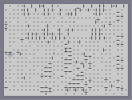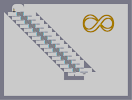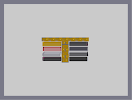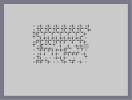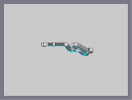V W Another Frustarating Maze Infinity Myspace Buttons Another, yet less frustrating, maze. Jag Test

Pages: (2) [ 1 ] 2

### ya...

And the dragon is at 4 now. =(

### HAHAHAHAHAHA

This is one of the only maps to "survive with a 5" for more than 1 year.
Still rocks

5/5

### oh my jesus

* faints *

and put a ninja and played it at 700 f/ms lol

.

5/5aved

### Holy fuck.

That's the only way I can describe this. Jesus. O_O
wow ur good at writing code

### thanks

This was full z snapping, however the entire code was written in notepad.
I could never finish it using only the mouse.

### that's just so good

hmm. afternoon dragon step 4 is better but it uses like the normal art technics like z-snapping mines filling them with c-snap.
this is full z snap (am i right?) and only thwumps and bounce blocks. possibly the only art i've seen with bounce blocks is afternoon dragon step 4 that i can remember. never though have i seen a good n-art made from thwumps.
5/5 not faved though cause i will always remember this n-art.

~teraza

btw, i've probably already commented idk.

thanks!

### um

he means u

ur the best n-art creator

### nart creator?

i have no program that helps me generate the code.

I just had lost of patience and free time.

I may start back up, but im havin too much fun drawing pics in the powder game where there are more than 2 colors to use for pixels.

### WOW.

NOW BEST NART CREATOR!!! :)

/bandwagon

.

.

### Awesome...

I reckon this is the greatest N-Art ever to be posted to NUMA! Good work.

5aved.

### Haha!

I love how Incluye has no knowledge of cars.

### i wouldnt be able to do that

if i even had the patience. quite an eye for detail you have raven. 5/5

### WTF?!?

U have no maps, and who r u callin' a douche?

The only person who was really mad at me had a pretty good map, but u have none.

Watch what u say before you post.

### douche....

dude check out my only map. its pretty cool

rate it and comment on it
this is the best n art i have ever seen. so much detail!!!

10/5

### NEAH!!!

how much free time do you have?/how long does it tame to load while making/if a ned was put in there, hoe much would it lagg?/i should stop now!

### I love you

you have proved to me that all modern N-art is not crap. I have not seen much good lately. mrgy seems to have retired from art too :(
thank you
\$5 for you and your cat.
5. This is awesome.
Also, pushing the guy who spammed the periods off the map.

beautiful

### oops

did i say 4/5, i meant 5/5

### 4/5

i saw this map a week ago, looked and the thumbnail, thought to myself "well that looks stupid", and moved on. I should have looked at the full size thing because this is
AWESOME!!

NICE ART

This is amazing

### Click the ninja next to the map name,

that's how you fave it.

### Incredible

Now how do you fave?

### One thing

I must say, awesome job with the stroke on the blue lines, I would much rather make this a conventional way, but you sir have patience. 5/5

### Safe

is slang for good, if that's what you meant.

What?!?

.